Include Top

# Summary of Features in General Linear Model

Statistical Tools > General Linear Model > Fit General Linear Model

Extends Advanced Multiple Regression to include:

• Fixed and Random Factors
• Nested Factors
• Covariates (can be Nested)
• For Random or Mixed Random/Fixed Factors with a balanced design, the ANOVA and Variance Components (VC) report is given based on Expected Mean Squares. VC confidence intervals using Restricted Maximum Likelihood (REML) are included.
• If the design is unbalanced or model is non-hierarchical, REML is used to compute the VC values and confidence intervals. Fixed Effects Tests are based on Satterthwaite approximation degrees of freedom.
• Main Effects with Confidence Intervals and Interaction Plots of Fitted Means for Non-Nested Fixed Factors
• Tukey and Fisher Pairwise Comparison of Means for Non-Nested Fixed Factors
• Predicted Response Calculator

Statistical Tools > General Linear Model > GLM Multiple Response Optimization

• Multiple Response Optimization for Nested or Non-Nested Fixed Factors

For details on the statistical methods, formulas and references, see the Appendix: General Linear Model. Multiple Response Optimization is introduced in Design of Experiments: Part F – Multiple Response Optimization with Advanced Multiple Regression. Note that if a factor is nested in one model and used in another, it must be nested the same way for all models.

Case study examples using GLM will be used to demonstrate the analysis of:

• Fixed Factors with Nested Variable
• Sources of Variation Study
• Classical Gage R&R
• Gage R&R Study with Operator as Fixed Factor
• Destructive (Nested) Gage R&R
• Expanded Gage R&R
• Unbalanced Nested Factorial Experimentwith Fixed and Random Factors

For further reading, the following book is recommended:

Montgomery, D.C. (2020). Design and Analysis of Experiments, 10th Edition, John Wiley & Sons. See chapter 13 "Experiments with Random Factors" and chapter 14 "Nested and Split-Plot Designs".

# General Linear Model Dialogs and Options

Fit General Linear Model Dialog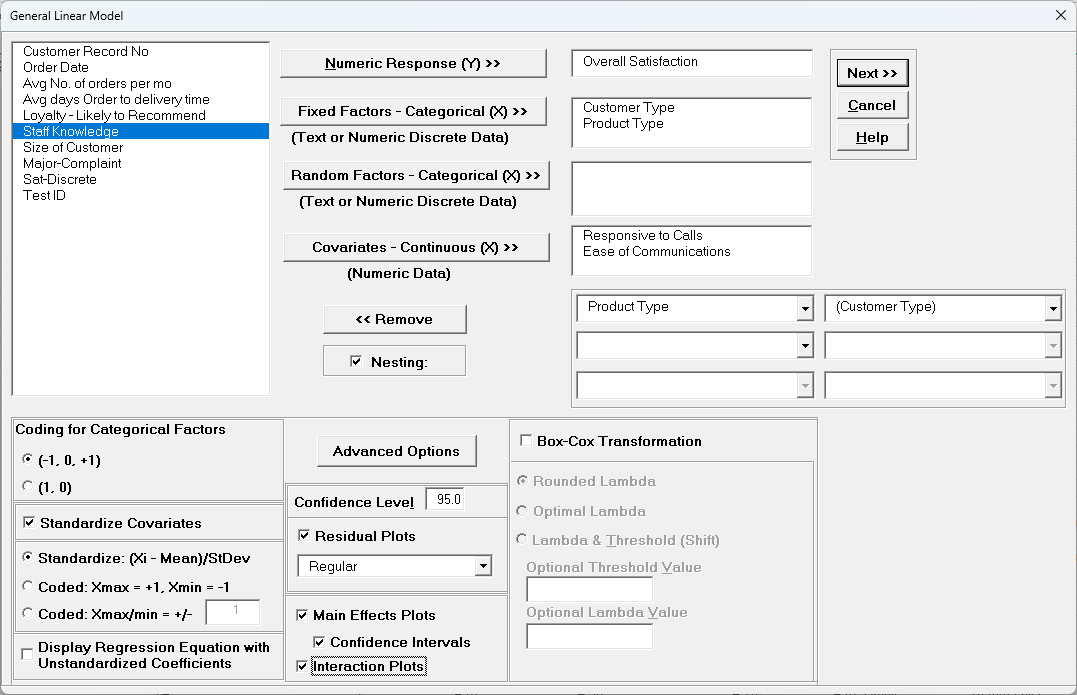• Numeric Response (Y) - select the response variable. Only one response may be selected at a time, but you can use Recall SigmaXL Dialog or Press F3 to repeat an analysis with different options or to select a different response. The regression reports will be created on sheetsGLM1 - Model Y1name, GLM2 - Model Y2name, etc., but truncated to fit the 31-character limit for Excel sheet names.

• Fixed Factors - Categorical (X) - select fixed categorical predictors. A fixed factor includes data for all levels of interest. Note, in previous analysis using categorical predictors for One & Two-Way ANOVA and Multiple Regression, the factors were assumed to be fixed.

• Random Factors - Categorical (X) - select random categorical predictors. A random factor has levels that are randomly sampled from a larger number of possible levels but interest is in all possible levels. If a Random Factor is selected, Advanced Options and Confidence Intervals for Main Effects Plots are greyed out. A variance components report will be produced in sheet GLM# - VarComp Yname. Note that the GLM regression report treats random factors as fixed. ANOVA for Predictors, Pareto Charts and CI/PI for Predicted Response Calculator are not available in the regression report. See the variance components report for analysis of random or random/fixed factors. Confidence Intervals for Main Effects Plots, Stepwise/Best Subsets Regression, K-Fold Cross Validation, and Pairwise Comparison of Means for Fixed Factors are not available.

• For Fixed and Random Factors, numeric variables can be used but they will be converted to text and an underscore "_" will be appended to the number. If there are more than 50 unique levels, a warning message is given. Typically, this occurs when the user has incorrectly selected a continuous variable as categorical. Note that the character "*" cannot be in the name as this is used to denote a cross product term and will be error trapped. Parenthesis characters "(" and ")" will be converted to underscore "_" since these are used to denote a nested term.

• Covariates - Continuous (X) - select continuous numeric variable of interest. Selections with data as text are error trapped. Note that the character "*" cannot be in the Covariate name as this is used to denote a cross product term and will be error trapped. Parenthesis characters "(" and ")" will be converted to underscore "_" since these are used to denote a nested term.

• Nesting - check to include nested terms in the model. The left-side combo dropdown selection can be a Factor or Covariate. The right-sidedropdown selection is a Factor. This will create a term with the notation A(B) where A is nested in B. For the example above, that would produce Product Type(Customer Type). The graphical representation of this is: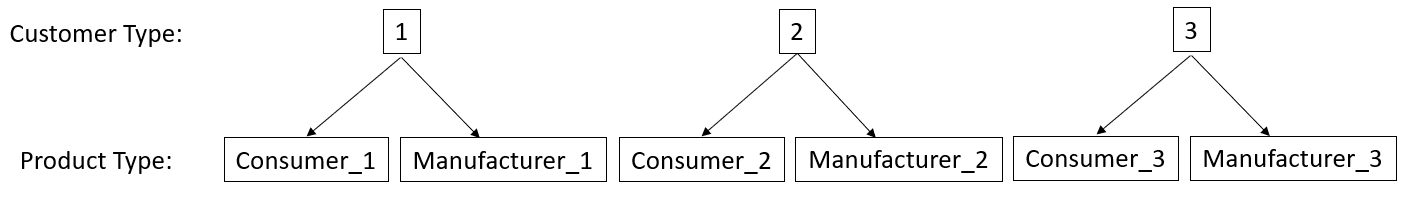The product type "Consumer_1" is unique to Customer Type 1, etc.

Another example of a nested term would be Operator(Location) where the operators in each plant location are different: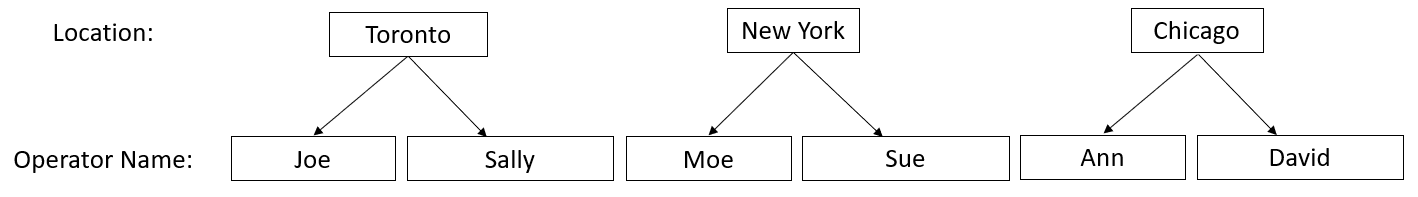A maximum of three levels of nesting are permitted with up to four factors. In the model, this would appear as "D, C(D), B(C(D)), A(B(C(D)))" or "A, B(A), C(B(A)), D(C(B(A)))" depending on the nesting assignment. One should exercise caution when using three levels of nesting as the number of coefficients in the model is equal to the number of factor combinations = levelsA*levelsB*levelsC*levelsD.

A factor cannot be nested within itself, either directly or indirectly, so model "A(B), B(A)" or "A(B), B(C), C(A)" would be illegal.

Note, "B(A), C(A)" is legal, but "A(B), A(C)" is illegal. A workaround to this limitation would be to create a new factor "B_C" by concatenating the levels of B and C and using "A(B_C)".

If B is nested in A, or A nested in B, i.e., B(A) or A(B), the interaction term A*B is not permitted. SigmaXL automatically excludes these interactions in the Specify Model Terms dialog. Interactions involving A or B with other factors are permitted.

Term hierarchy in a model is recommended, but not required.

For further information on nesting see the Appendix: Nested Factors and Coding and Nested Covariates.

• Coding for Categorical Factors (-1, 0, +1), also known as effects coding, estimates the difference between each factor level mean and the overall mean. It results in lower multicollinearity VIF scores than (1, 0) coding. The reference level is the last alpha-numerically sorted level and is hidden in the Parameter Estimates table.

• Coding for Categorical Factors (1, 0), also known as dummy coding, is the coding scheme typically used for categorical predictors in a regression analysis. The hidden reference value is the first alpha-numerically sorted level.

• Standardize Continuous Predictors with Standardize: (Xi - Mean)/Stdev will convert continuous predictors to Z-scores. This has two benefits: the predictors are scaled to the same units so coefficients can be meaningfully compared, and it dramatically reduces the multicollinearity VIF scores when interactions and/or quadratic terms are specified.

• Standardize Continuous Predictors with Coded: Xmax = +1, Xmin = -1 scales the continuous predictors so that Xmax is set to +1 and Xmin is set to -1. This is particularly useful for analyzing data from a full or fractional-factorial design of experiments.

• Standardize Continuous Predictors with Coded: Xmax/Xmin = +/- value scales the continuous predictors so that Xmax is set to +value and Xmin is set to -value. This is particularly useful if one is analyzing data from a response surface design of experiments, where value is set to the alpha axial value such as 1.414 for a two-factor rotatable design.

• Display Regression Equation with Unstandardized Coefficients displays the prediction equation with unstandardized/uncoded coefficients but the Parameter Estimates table will still show the standardized coefficients. This format is easier to interpret since there is only one coefficient value for each predictor.

• Confidence Level is used to determine what alpha value is used to highlight P-Values in red, the significance reference line in the Pareto Chart of Standardized Effects, and the percent confidence and prediction interval used in the Predicted Response Calculator.

• Residual Plots Regular display the raw residuals (Y - Ŷ) with a Histogram, Normal Probability Plot, Residuals vs Data Order, Residuals vs Predicted Values, Residuals vs Continuous Predictors and Residuals vs Categorical Predictors.

• Residual Plots Standardized display the residuals, divided by an estimate of its standard deviation. This makes it easier to detect outliers. Standardized residuals greater than 3 and less than -3 are considered large (these outliers are highlighted in red).

• Residual Plots Studentized (Deleted t)display studentized deleted residuals which are computed in the same way that standardized residuals are, except that the i th observation is removed before performing the regression fit. This prevents the ith observation from influencing the regression model, resulting in unusual observations being more likely to stand out.

• The Residuals report is provided on a separate sheet and includes a table with all residual types to the left of the plots. Other diagnostic measures included, but not plotted are Cook's Distance (Influence), Leverage and DFITS. Leverage is a measure of how far an individual X predictor value deviates from its mean. High leverage points can potentially have a strong effect on the estimate of regression coefficients. Leverage values fall between 0 and 1. Cook's distance and DFITS are overall measures of influence. An observation is said to be influential if removing the observation substantially changes the estimate of model coefficients. Cook's distance can be thought of as the product of leverage and the standardized residual squared; DFITS as the product of leverage and the studentized residual. These diagnostic measures can be manually plotted using a Run Chart to identify unusually large values. Commonly used rough cutoff criterion for Cook's distance are: > 0.5, potentially influential and > 1, likely influential. A more accurate cutoff is the median of the F distribution: > F(0.5,p,n-p), where n is the sample size and p is the number of terms in the model design matrix, including the constant. A commonly used cutoff criterion for the absolute value of DFITS is:>2(p/n)). An observation that is an outlier and influential should be examined for measurement error or possible assignable cause. You could also try refitting the model excluding that observation to assess the influence.

• The Residuals report also includes a table to the right of the plots with the stored model design matrix and residuals. This can be used to manually create additional residual plots such as residuals versus interaction or quadratic terms.

• Tip: For large datsets (> 1K) you may want to uncheck the Residual Plots in order to speed up the analysis.

• Main Effects Plots with Confidence Intervals and Interaction Plots use fitted means, not data means. If an interaction term is not in the model, the interaction plot is still displayed, but it is shaded grey. If the model is not hierarchical, these plots are not displayed.

• Box-Cox Transformation with Rounded Lambda will solve for an optimal lambda and is rounded to the nearest value of: -5, -4, -3, -2, -1, -0.5, 0, 0.5, 1, 2, 3, 4, 5. A 0 denotes a Ln(Y) transformation, 0.5 is the SQRT(Y), and 1 is untransformed. Threshold (Shift) is computed automatically if the response data includes 0 or negative values, otherwise it is 0. Note that the threshold is subtracted from the data so the value will be negative in order to provide positive response values. Solving lambda is also supported in Stepwise Regression. The reported Parameter Estimates, Model Summary, Information Criteria, Validation, Test Statistics and Residuals are for the Box-Cox transformed response. The Predicted Response Calculator automatically applies an inverse transformation so that the predicted response, confidence and prediction intervals are given in the original untransformed units. Note, Lambda is solved to normalize the regression residuals, not the raw data. It is solved using the classical Box-Cox formula but the actual transformation uses a simple power transformation.

• Box-Cox Transformation with Optimal Lambda uses the range of -5 to +5 for Lambda. Threshold is computed automatically if the response data includes 0 or negative values.

• Box-Cox Transformation with Lambda & Threshold(Shift) allows the user to specify Lambda and Threshold. Threshold is typically 0, but if the response data includes 0 or negative values, a negative threshold value should be entered, such that when subtracted from the data, results in positive response values.

• General Linear Model Options Dialog (not available if Random Factor selected)• Assume Constant Variance/No AC (no autocorrelation in the residuals), if unchecked, SigmaXL will use either White robust standard errors for non-constant variance or Newey-West robust standard errors for non-constant variance with autocorrelation. If either of the Durbin-Watson P-Values are < .05 (i.e., significant positive or negative autocorrelation), Newey-West for Lag 1 is used, otherwise White HC3 is used. This will affect SE Coefficients, P-Values, ANOVA F and P-Values, and Prediction CI/PI. ANOVA F and P-Values are Wald estimates. ANOVA SS Type I Table and Pareto Charts are not available. Note: Stepwise P-Values are not adjusted.

• Term ANOVA Sum of Squares with Adjusted (Type III) provides a detailed ANOVA table for continuous and categorical predictors. Adjusted Type III is the reduction in the error sum of squares (SS) when the term is added to a model that contains all the remaining terms. Note, categorical terms are considered as a group, unlike the parameter estimates table which uses coding.

• Term ANOVA Sum of Squares with Sequential (Type I) provides a detailed ANOVA table for continuous and categorical predictors. Sequential Type I is the reduction in the error sum of squares (SS) when a term is added to a model that contains only the terms before it. This is affected by the order that they are enteredin the model, so the user must be careful to specify model terms in the order of importance based on process knowledge. Note, if the terms are orthogonal then Type III and Type I SS will be the same.

• R-Square Pareto Chart displays a Pareto chart of term R-Square values (100*SS term/SStotal). A separate Pareto Chart is produced for Type III and Type I SS. If there is only one predictor term, a Pareto Chart is not displayed.

• Standardized Effect Pareto Chart displays a Pareto chart of term T values (=T.INV(1-P/2,df error)). A separate Pareto Chart is produced for Type III and Type I SS. A significance reference line is included (=T.INV(1-alpha/2,dferror)).

• K-Fold Cross Validation: In K-Fold cross-validation, the data is randomly partitioned into K (approximately equal) subsets. The model coefficients are estimated using K-1 partitions, i.e., (100*(K-1)/K)% of the data - the training set, and then statistical metrics are evaluated on the remaining data - the validation set. This is repeated for each of the K-Fold validation sets with R-Square K-Fold and S (Standard Deviation) K-Fold calculated as an average across the K samples, which results in a more accurate estimate of model prediction performance. The default K=10 is a popular choice, but some practitioners prefer K=5. Note that the final model parameter coefficients are based on all of the data, so K-Fold Cross Validation is used strictly to obtain R-Square K-Fold and S K-Fold. The fixed seed allows for replicable results, but the user may wish to re-run the analysis with a different seed a few times to see how much variation occurs in R-Square K-Fold and S K-Fold. If categorical predictors are used and the training sample does not include all of the levels, the K-Fold statistics cannot be computed.

• Pairwise Comparison of Means for Fixed Factors: Tukey or Fisher. Pairwise comparisons of means examines the difference between all combinations of the estimated means for each category of a factor, along with the standard error and confidence band for the difference. Tukey provides protection against false positives due to multiple comparisons so is the default selection. If the model is not hierarchical, the pairwise comparison report is not available. For further information see the Appendix: Pairwise Comparison of Means for Fixed Factors.

• Stepwise/Best Subsets Regression with Forward/Backward Stepwise: Starting with an empty model, terms are added or removed from the model, one at a time, until all variables in the model have p-values that are less than (or equal to) the specified Alpha-to-Remove and all variables that are not in the model have p-values greater than the specified in Alpha-to-Enter. The stepwise process either adds the term which is most significant (largest F statistic, smallest p-value), or removes the term that is least significant (smallest F statistic, largest p-value). It does not consider all possible regression models. The independent variables can be continuous and/or categorical. A categorical predictor is treated as a group, so is either all in or all out.

• Stepwise/Best Subsets Regression with Forward Selection: Starting with an empty model, the most significant terms are added to the model, one at a time, until all variables that are not in the model have p-values greater than the specified in Alpha-to-Enter. Terms that are in the model are not removed regardless of p-value. Alternatively, criterion-based selection may be used. The most significant terms are added, one at a time, while at each stage the value of a measure, such as AICc or R-Square is monitored. If a minimum AICc is observed at step i, and this remains the minimum after 10 additional steps (or the model includes all terms), then the model at the minimum AICc is selected. If a maximum R-Square is observed at step i, and this remains the maximum after 10 additional steps, then the model with the maximum R-Square is selected. Criterion options are: AICc, BIC, R-Square Adjusted, R-Square Predicted and R-Square K-Fold. AICc is the Akaike Information Criterion corrected for small sample sizes, BIC is the Bayesian Information Criterion. For details on these metrics, see the Appendix: Advanced Multiple Regression. Note that for R-Square K-Fold, the F-statistic to decide which term to enter is based on all of the data. The K-Fold model is computed using the specified model, but a subset of the data is used as training data to estimate parameters and R-square is calculated using the out-of-sample validation data. As with forward/backward stepwise, the independent variables can be continuous and/or categorical. A categorical predictor is treated as a group, so is either all in or all out.

• Stepwise/Best Subsets Regression with Backward Elimination: Starting with all terms in the model, the least significant terms are removed from the model, one at a time, until all variablesin the model have p-values that are less than (or equal to) the specified Alpha-to-Remove. Terms that are removed from the model are not entered again regardless of p-value. Alternatively, criterion-based selection may be used, as described above, but the least significant terms are removed, one at a time. It stops after 10 additional steps or if the model includes only one term. As with forward/backward stepwise, the independent variables can be continuous and/or categorical. A categorical predictor is treated as a group, so is either all in or all out.

• Stepwise/Best Subsets Regression with Best Subsets: With Best Subsets, for any given model with p terms, there are 2p - 1 possible combinations (non-hierarchical models). A criterion such as AICc is specified, and the model which results in the minimum AICc is selected. If p ≤ 15, all possible combinations are explored - this is called exhaustive. Otherwise, the best model is derived using discrete optimization with the powerful MIDACO Solver (Mixed Integer Distributed Ant Colony Optimization). Start values are obtained using forward selection with the AICc criterion. MIDACO does not guarantee a best solution as we have in exhaustive, but will be close to best, even for hundreds of terms! Best Subsets Criterion options are: AICc, BIC and R-Square Adjusted. R-Square Predicted and R-Square K-Fold are not feasible as criterion here due to the computation times, but they are reported on the best selected models. Best Subsets report options are: Best For Each # of Pred (default) or Best Overall. Best For Each # of Predictors provides the most information but takes longer to compute than Best Overall. The user may specify how many models to include (per # predictors or overall) in the report, with the default = 1. The default Max Time (sec) = 300 is the maximum total computation time allotted for either option.The independent variables can be continuous and/or categorical. A categorical predictor is treated as a group, so is either all in or all out.

• Stepwise/Best Subsets Regression - Hierarchical: The Hierarchical option constrains the model so that all lower order terms that comprise the higher order terms are included in the model. This is checked by default. In Forward/Backward Stepwise and Forward Selection, a hierarchical model is required at each step, but extra terms can enter to maintain hierarchy. For Backward Elimination and Best Subsets, extra terms are not permitted.

• Saturated Model Pseudo Standard Error (Lenth's PSE): For saturated models with dferror = 0, Lenth's method is used compute a pseudo standard error. For each term, a t ratio is computed by dividing the coefficient by the PSE. Since the distribution of the t ratio is not analytic, the probability is evaluated using Monte Carlo simulation. Student TP-Values are also available for comparison purposes.Lenth's PSE in the SigmaXL DOE Templates and DOE Analysis use Student T P-Values.

Tip: There are a lot of options here, giving the user flexibility for model refinement, but this can also be overwhelming to someone starting out with these tools. We recommend using the following settings for Stepwise/Best Subsets Regression:

1. Forward Selection, Criterion: AICc, BIC or R-Square Predicted, Hierarchical checked. This is fast and will build a model that minimizes AICc, BIC or maximizes R-Square Predicted. AICc or R-Square Predicted are recommended for the best model prediction accuracy, BIC is recommended for model parsimony. Note, however, this does not consider all possible models.

2. Best Subsets, 1 For Each # of Pred, Max Time (sec) = 300, Criterion: AICc or BIC, Hierarchical checked. This can be slow but gives a very useful report of the best model for each number of predictors in the model.

Specify Model Terms Dialog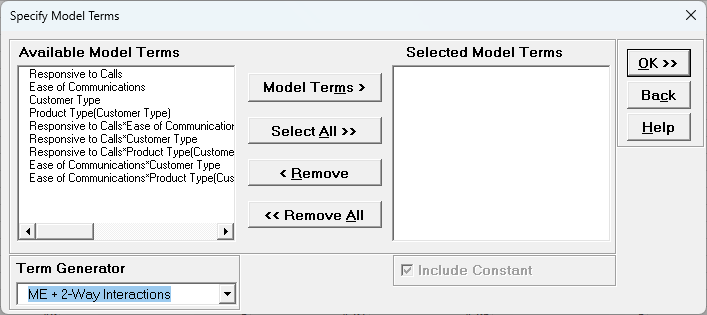• Term Generator - select any of the following to build a list of Available Model Terms:

• Main Effects - default - no change to original specified terms

• ME+ 2-Way Interactions - use this to include 2-way interactions in the model, for example, analyzing data from a Res IV or Res V fractional-factorial DOE. When specifying interactions or higher order terms, standardization of continuous predictors is highly recommended.

• ME + 2-Way Interactions + Quadratic - use this to include 2-way interactions andquadratic terms in the model, for example, analyzing data from a response surface DOE. Categorical terms will not be squared.

• ME + All Interactions - use this to include all possible interaction terms in the model, for example analyzing data from a full-factorial DOE.

• All up to 3-Way - use this to include 2-way interactions, quadratic, 3-way interactions, quadratic*main effect and cubic terms in the model. Categorical terms will not be squared or cubed.

• Model Terms: Select from highlighted Available Model Terms.

• Select All: Select all Available Model Terms. Caution, the number of selected terms can become quite large, especially for the last two options in the Term Generator. If more than 100 terms are selected, a warning is given after clicking OK: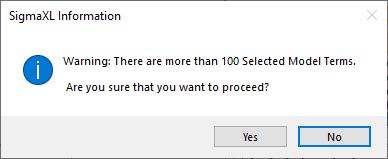• Include Constant: Always checked in GLM.

Tip: It is also important to ensure that the number of rows/observations are sufficient to estimate the number of selected model terms. A rule of thumb (excluding data from a designed experiment) is that for every term selected, there should be a minimum of 10 rows of data. This rule holds for potential terms used in stepwise and best subsets as well, otherwise one can easily produce a model that is highly significant but a meaningless model of noise. This is what Jim Frost calls Data Dredging in chapter 8 of his book Regression Analysis: An Intuitive Guide for Using and Interpreting Linear Models.

# Example 1: Fixed Factors with Nested Variable

1. Open Customer Data.xlsx. Click Sheet 1 Tab. Click SigmaXL > Statistical Tools > General Linear Model> Fit General Linear Model. If necessary, click Use Entire Data Table, click Next.

2. Select Overall Satisfaction, click Numeric Response (Y) >>, select Customer Type and Product Type, click Fixed Factors - Categorical (X) >>, select Responsive to Calls and Ease of Communications, click Covariates - Continuous (X) >>. Check Nesting. For the left-side drop-down "Select a Factor or Covariate", select Product Type. For the right-side drop-down "Select a Factor to Nest in:" select (Customer Type). We will use the default Coding for Categorical Predictors (-1,0, +1). Check Standardize Covariates with default option Standardize: (Xi-Mean)/StDev. Use the default Confidence Level = 95.0%. Regular Residual Plots are checked by default. Check Main Effects Plots with Confidence Intervals. Leave Interaction Plots and Box-Cox Transformation unchecked.Tip: If you have covariates (continuous predictors) and are planning to include interaction terms in the model, always ensure that Standardize Covariates is checked. This has two benefits: the covariates are scaled to the same units so coefficients can be meaningfully compared, and it dramatically reduces the multicollinearity VIF scores.

3. Click Advanced Options. We will use the default options as shown with K-Fold Cross Validation and Stepwise/Best Subsets Regression unchecked. Pairwise Comparison of Means for Fixed Factors with Tukey option is checked.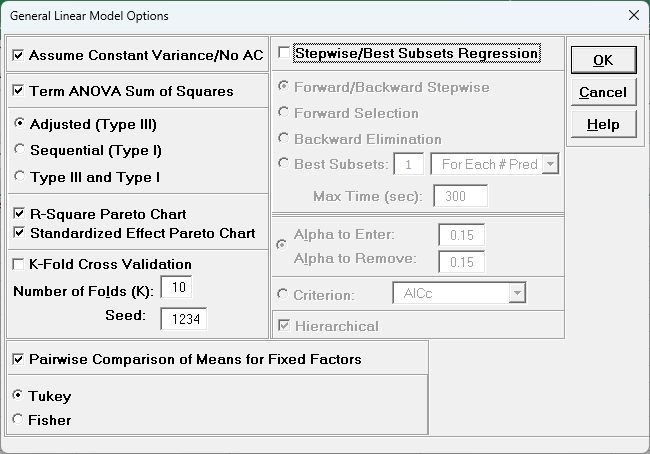4. Click OK. Click Next >>.

5. Using Term Generator, select ME + 2-Way Interactions. Select Responsive to Calls to Responsive to Calls*Ease of Communication. Click Model Terms >. Include Constant is always checked in General Linear Model.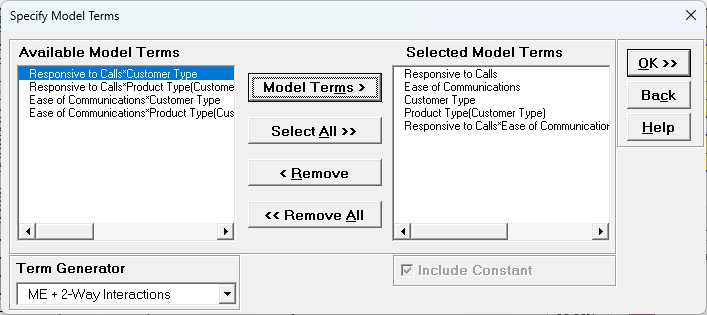Product Type(Customer Type) denotes that Product Type is nested within Customer Type. Note, this data is not actually nested, but we are using this as an example to demonstrate nesting with familiar data.

Term Generator, ME + 2-Way Interactions produces only legal two-way interactions, so Product Type*Customer Type is not available for selection. Based on previous regression analysis for Overall Satisfaction, we are only including the Responsive to Calls*Ease of Communications 2-way interaction.

6. Click OK>>. The General Linear Model report is given. The Parameter Estimates - Standardized and ANOVA tables are shown: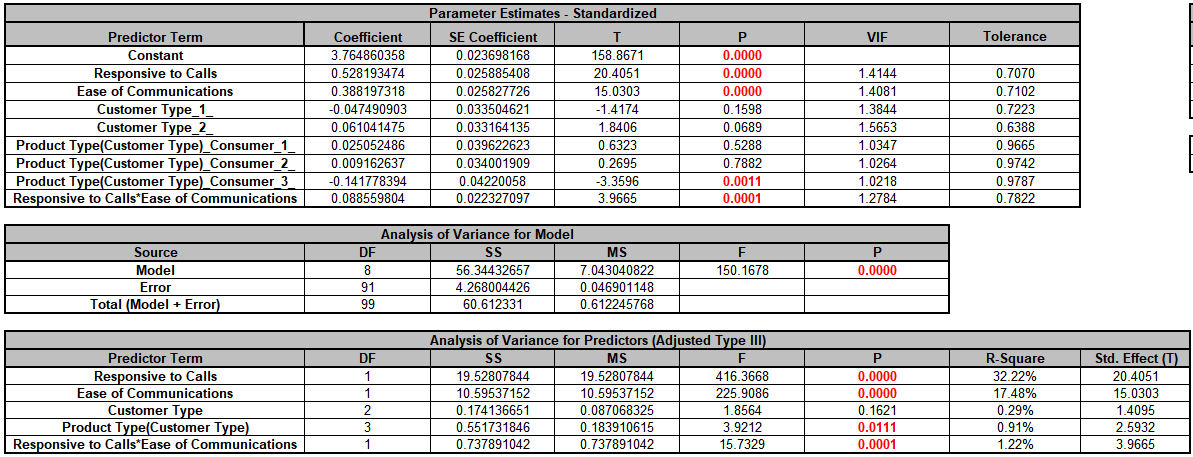With (-1, 0, 1) coding, the reference level is the last alpha-numerically sorted level. The hidden reference level for Customer Type is 3.

"Product Type(Customer Type)_Consumer_1_" denotes Product Type Consumer nested within Customer Type 1. The hidden reference level for Product Type is Manufacturer. For further information on nesting see the Appendix: Nested Factors and Coding.

The Analysis of Variance for Predictors (Adjusted Type III) table shows that Customer Type is not significant with P-Value = 0.162, but we will leave it in the model to maintain hierarchy since Product Type is nested within Customer Type. Product Type is significant with P-Value = 0.011.

7. The GLM Equation with standardized coefficients is:

General Linear Model: Overall Satisfaction = (3.76486)

+ (0.528193)*((Responsive_to_Calls-3.8644)/1.14029)

+ (0.388197)*((Ease_of_Communications-3.7481)/0.911361)

+ (-0.0474909)*(IF(Customer_Type="1_",1,0)+IF(Customer_Type="3_",-1,0))

+ (0.0610415)*(IF(Customer_Type="2_",1,0)+IF(Customer_Type="3_",-1,0))

+ (0.0250525)*(IF(Product_Type="Consumer",1,0) + IF(Product_Type="",-1,0) + IF(Product_Type="Manufacturer",-1,0))*IF(Customer_Type="1_",1,0)

+ (0.00916264)*(IF(Product_Type="Consumer",1,0) + IF(Product_Type="",-1,0) + IF(Product_Type="Manufacturer",-1,0))*IF(Customer_Type="2_",1,0)

+ (-0.141778)*(IF(Product_Type="Consumer",1,0) + IF(Product_Type="",-1,0) + IF(Product_Type="Manufacturer",-1,0))*IF(Customer_Type="3_",1,0)

+ (0.0885598)*((Responsive_to_Calls-3.8644)/1.14029)*((Ease_of_Communications-3.7481)/0.911361)

Note blanks and special characters in the predictor names are converted to the underscore character "_". The numeric Customer Type 1, 2, 3 has also been converted to text so appear as "1_", "2_", "3_".

For categorical predictors, IF statements are used.

This is the display version of the prediction equation given at cell L14(which has more precision for the coefficients and predictor names are converted to legal Excel range names by padding with the underscore "_" character). If the equation exceeds 8000 characters (Excel's legal limit for a formula is 8192), then a truncated version is displayed and cell L14does not show the formula.

8. Scroll to the Predicted Response Calculator. Enter Responsive to Calls and Ease of Communication values = 5 with Customer Type = 1_ and Product Type = Consumer from the dropdown lists to predict Overall Satisfaction including the 95% confidence interval for the long term mean and 95% prediction interval for individual values: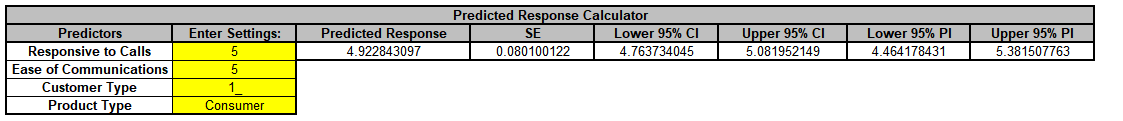9. Next, we will use SigmaXL's built in Optimizer. Scroll to view the Optimize Options: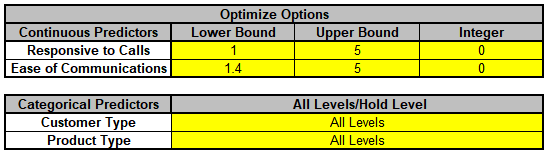Here we can constrain the continuous predictors and specify a level to use for optimization of the categorical predictors. If a continuous predictor is integer, change the Integer 0 to 1, and the Optimizer will return only integer values for that predictor.

We will leave the Optimize Option settings as is.

10. Scroll back to view the Goal setting and Optimize button. Select Goal = Maximize.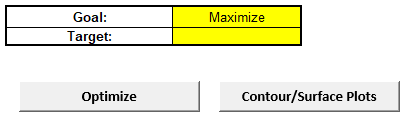The optimizer uses Multistart Nelder-Mead Simplex to solve for the desired response goal with given constraints. For more information see the Appendix: Single Response Optimization.

11. Click Optimize. The response solution and prompt to paste values into the Input Settings of the Predicted Response Calculator is given: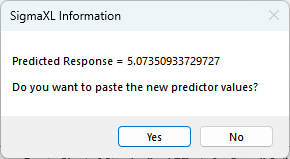12. Click Yes to paste the values.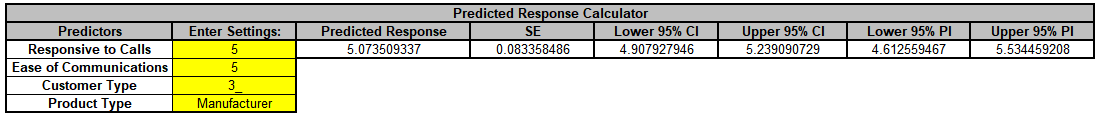The optimizer has selected Customer Type = 3_ and Product Type = Manufacturer to maximize the Overall Satisfaction predicted value.

Note that the optimizer does not test the validity of a nested combination, so if the goal is to optimize, it is best to use generic level names as done in this example. For Product Type, we use the generic levels "Consumer" and "Manufacturer", not "Consumer_Type1", "Consumer_Type2", "Consumer_Type3"; "Manufacturer_Type1", "Manufacturer_Type2", "Manufacturer_Type3".

13. Click on Sheet GLM1 - Pair Comp. The Tukey Pairwise Comparison of Means for Customer Type is shown.Pairwise comparisons of means examines the difference between all combinations of the estimated means for each category of a factor, along with the standard error and confidence band for the difference. Tukey provides protection against false positives due to multiple comparisons. Nested factors are not shown in the pairwise comparison report, so Product Type is not included here. For further information see the Appendix: Pairwise Comparison of Means for Fixed Factors.

As expected from the ANOVA report, none of the pairwise comparisons are significant.

Click on Sheet GLM1 - Plots. The Main Effects Plots with 95% Confidence Intervals are shown.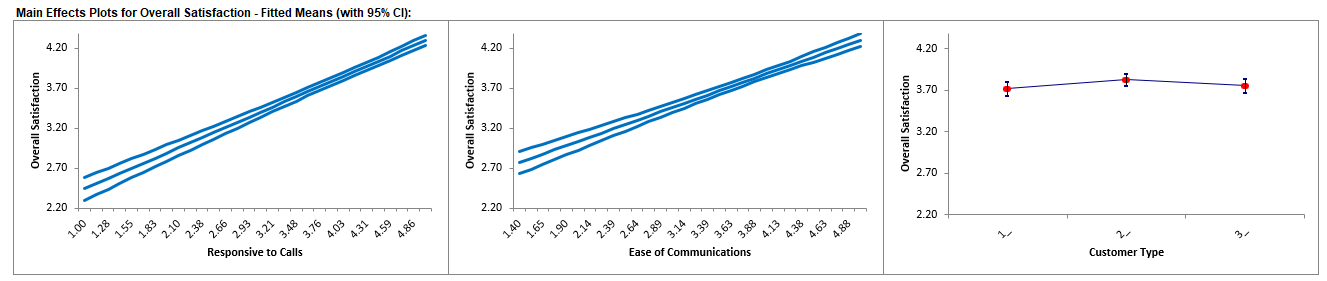These are based on Fitted Means as predicted by the model, not Data Means. They use the predicted value for the response versus input predictor value, while holding all other variables constant. Continuous are held at their respective means and categorical are weighted equally.

Here we see that Responsive to Calls has the steepest slope followed by Ease of Communications. As expected, Customer Type does not appear to be an important factor.

# Example 2: Sources of Variation Study

Open We will now reanalyze the Multi-Vari data presented in Multi-Vari Charts. The Multi-Vari chart was used to identify dominant Sources of Variation (SOV), with the three major families of variation being Within Unit, Between Unit, and Temporal (Over Time).

Dominant Within Unit Source of Variation: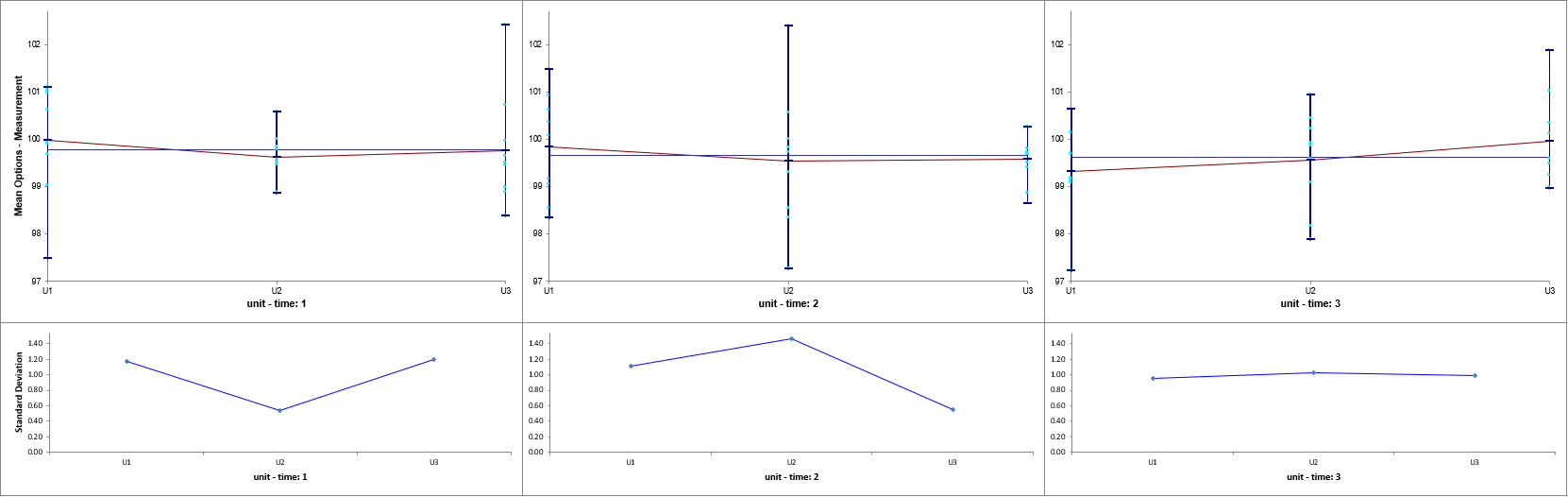Dominant Between Unit Source of Variation: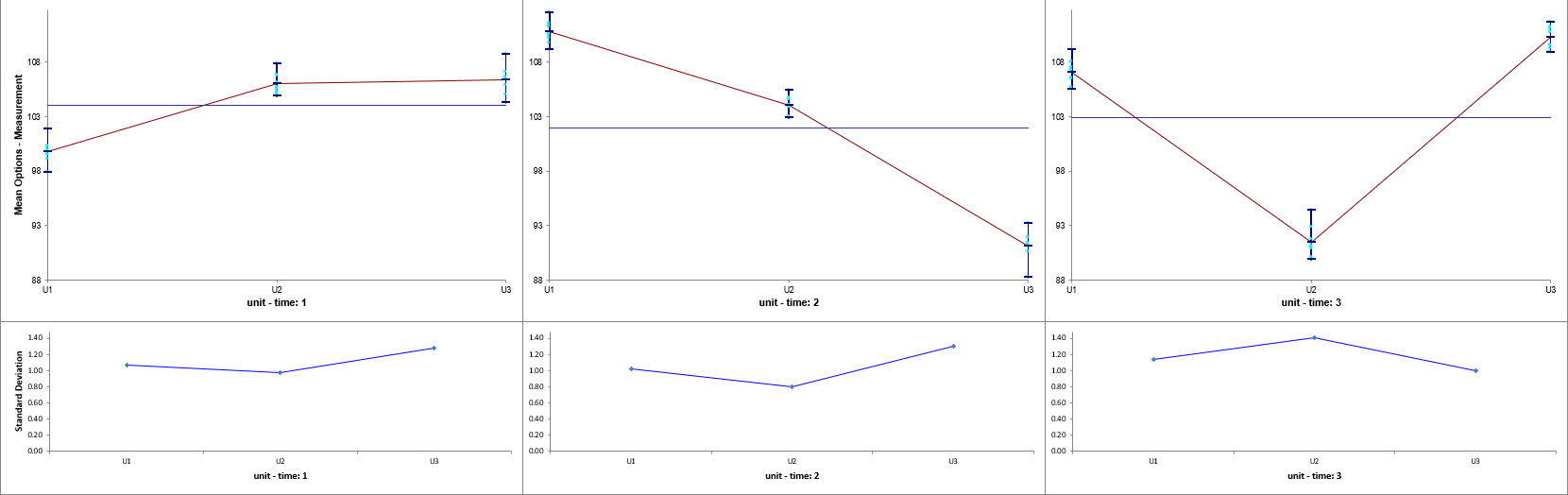Dominant Over Time Source of Variation:Unit and Time are Random Factors and Unit is nested within Time. The Variance Components will be calculated to quantify the percent contribution to total variation for each factor.

1. Open Multi-Vari Data.xlsx, click Sheet Within. Click SigmaXL > Statistical Tools > General Linear Model > Fit General Linear Model. If necessary, click Use Entire Data Table, click Next.

2. Select Measurement, click Numeric Response (Y) >>, select time, click Random Factors - Categorical (X) >>, select unit, click Random Factors - Categorical (X) >>. Check Nesting. For the left-side drop-down Select a Factor or Covariate, select unit. For the right-side drop-down Select a Factor to Nest in:, select (time). We will use the default Coding for Categorical Predictors (-1,0, +1) and default Confidence Level = 95.0%. Leave Residual Plots, Main Effects Plots, Interaction Plots and Box-Cox Transformation unchecked. Advanced Options are not available with Random Factors.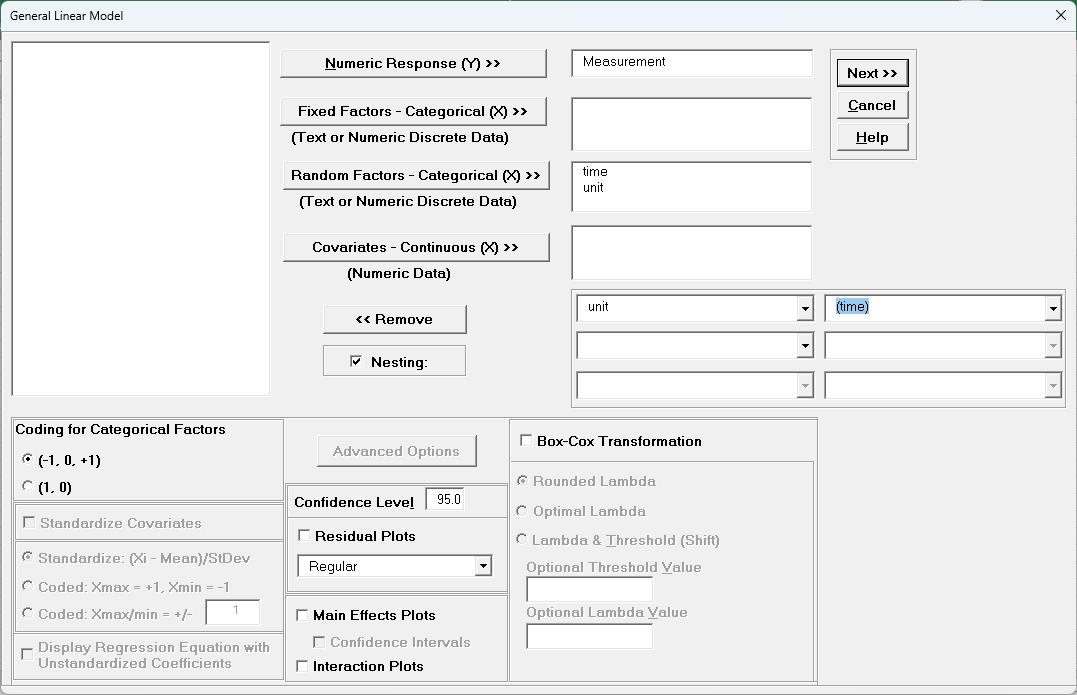3. Click Next >>.

4. Leave Term Generator as Main Effects. Click Select All >>. (If the order is reversed, select time, click Model Terms >, select unit(time), click Model Terms >.)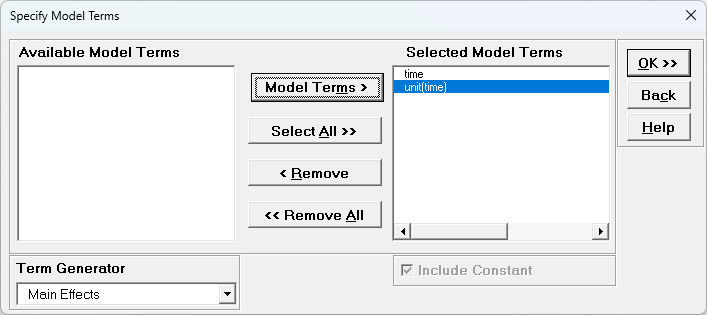The term unit(time) denotes that unit is nested within time. Since time is the top level of the nesting, we place it in the model first.

5. Click OK >>. The Variance Components report is given: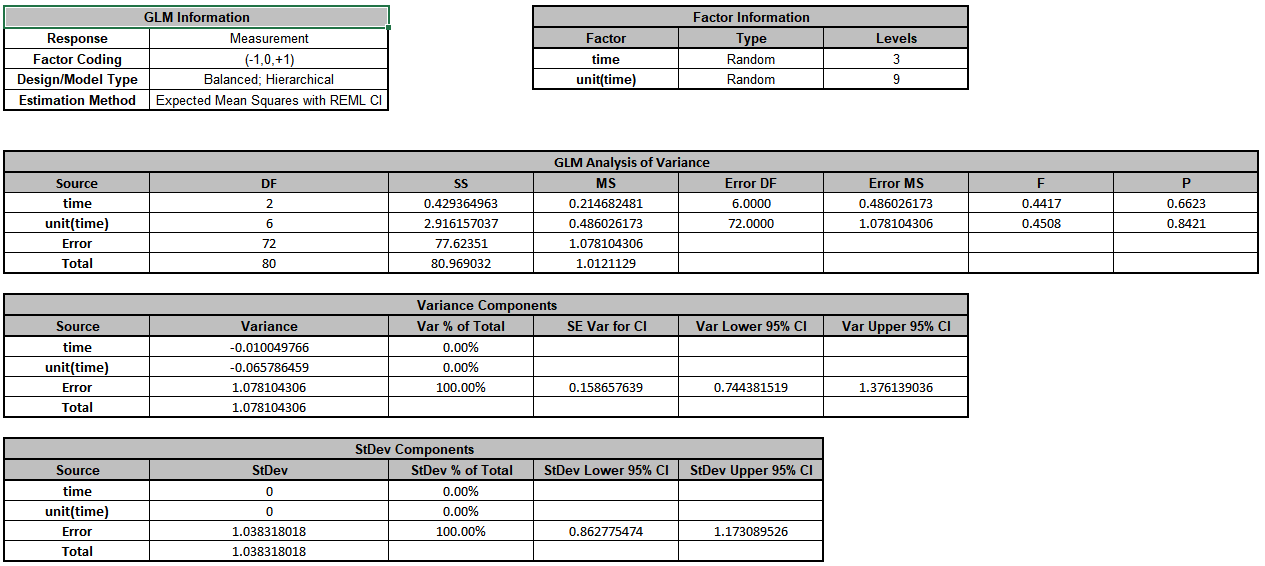The Error term in the variance components table is the Within Unit variation. It is contributing to 100% of the total variation. The REML 95% confidence interval for this variance component is also given.

The terms time and unit(time) have negative Variance Component values, so are treated as 0. The ANOVA table shows that both terms are not significant random factors (alpha = 0.05). Standard Deviation components are provided for convenience and useful in Measurement Systems Analysis, but they will not be discussed here.

The GLM Model sheet is the regression analysis but we will not review that here as our focus is on the variance components analysis. Note that the regression analysis treats random factors as fixed.

6. Now click sheet Between. Repeat steps 1 to 5 to produce the Variance Components report: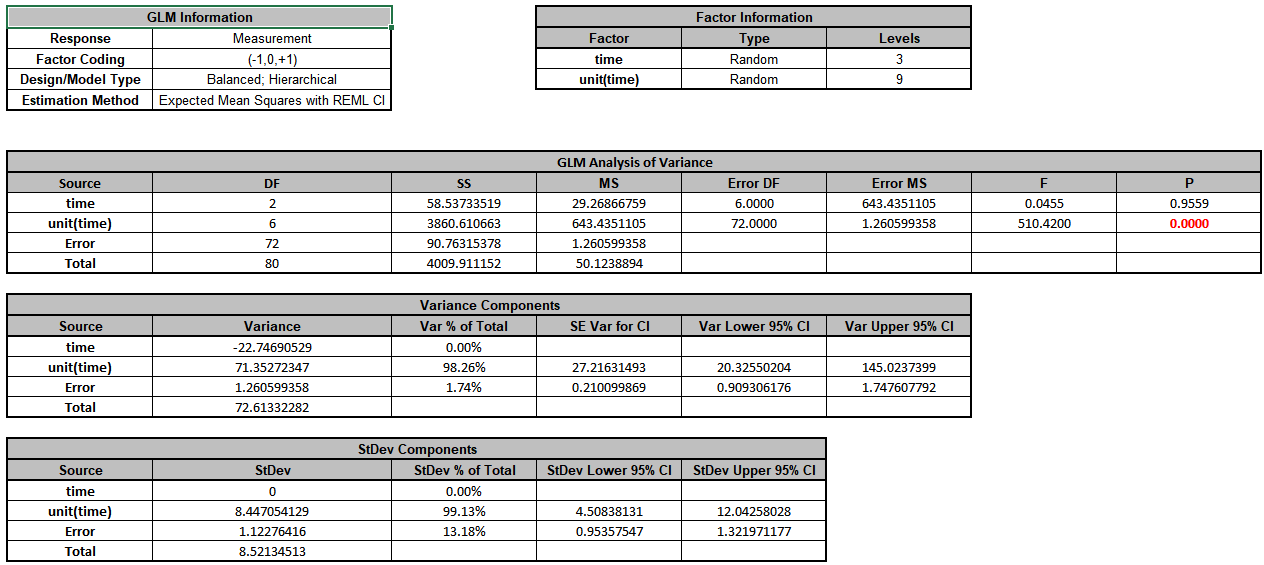The unit(time) term in the variance components table is the Between Unit variation. It is contributing to 98.3% of the total variation. The Within Unit Error term is contributing to 1.7% of the total variation. The REML 95% confidence interval for these variance components are also given.

The term time has a negative Variance Component value, so is treated as 0. The ANOVA table shows that time is not a significant random factor, but unit(time) is significant (alpha = 0.05).

7. Now click sheet Over Time. Repeat steps 1 to 5 to produce the Variance Components report:The time term in the variance components table is the Over Time variation. It is contributing to 98.5% of the total variation. The Between Unit unit(time) term is contributing to 0.6% of the total variation. The Within Unit Error term is contributing to 0.9% of the total variation. The REML 95% confidence interval for these variance components are also given. Note that the confidence interval for time is very wide. This is due to only having 3 levels. If possible, additional time value data should be collected in order to reduce the uncertainty of the variance component value.

The ANOVA table shows that time and unit(time) are significant random factors (alpha = 0.05).

# Example 3: Classical Gage R&R Study

We will now reanalyze the AIAG Gage R&R data presented in Analyze Gage R&R (Crossed). The Gage R&R Study Report was given as: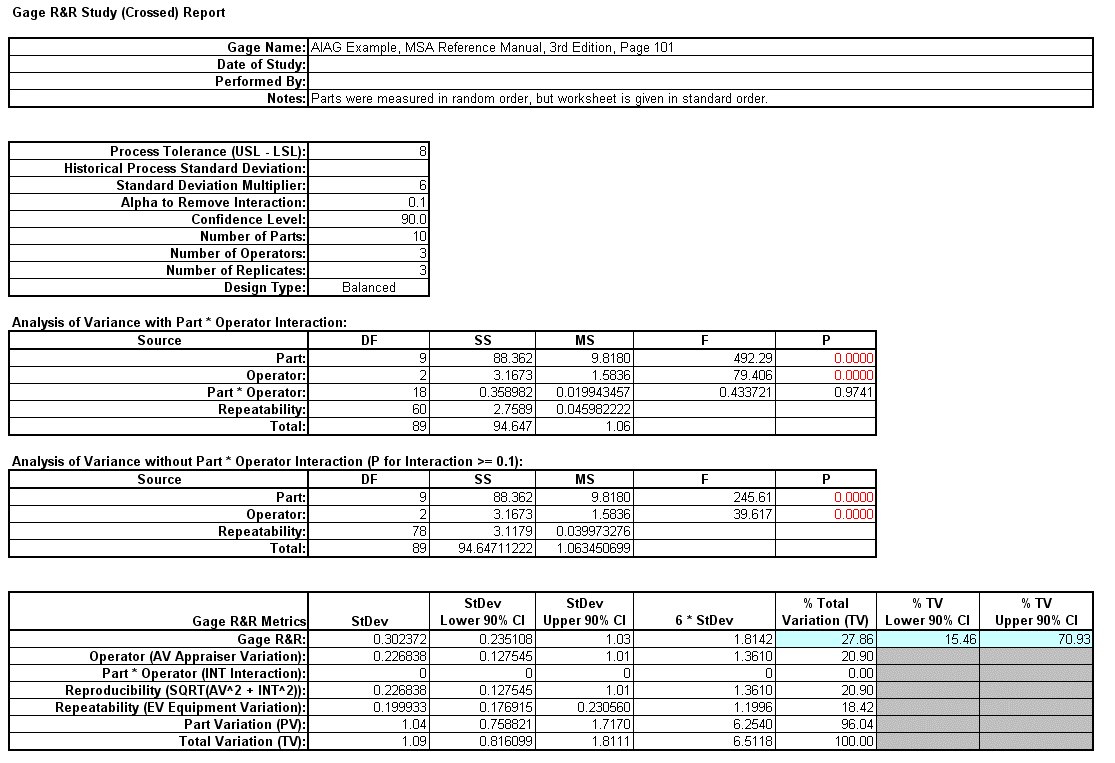1. Open the file Gage RR - AIAG.xlsx. This is an example from the Automotive Industry Action Group (AIAG) MSA Reference Manual, 3rd Edition, page 101. Note that parts were measured in random order, but the worksheet is given in standard order. Preselect the worksheet data including column headings. Click SigmaXL > Statistical Tools > General Linear Model > Fit General Linear Model. Click Next >>.

2. Select Measurement, click Numeric Response (Y) >>, select Part and Operator, click Random Factors - Categorical (X) >>. Leave Nesting unchecked. We will use the default Coding for Categorical Predictors (-1,0, +1). Set the Confidence Level = 90% to match the previous Gage R&R analysis. Leave Residual Plots, Main Effects Plots, Interaction Plots and Box-Cox Transformation unchecked. Advanced Options are not available with Random Factors.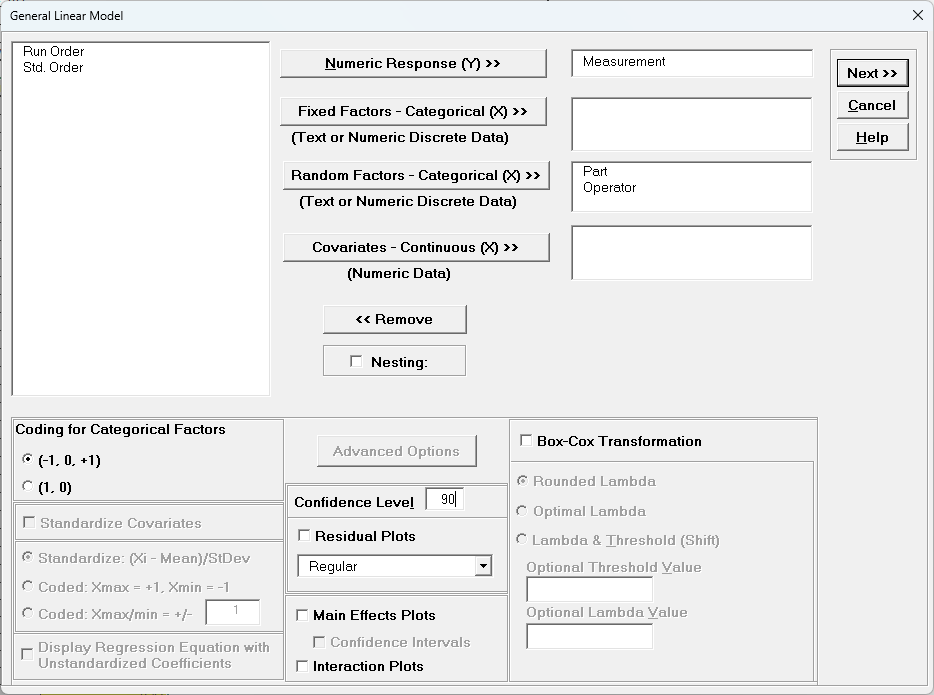3. Click Next >>.

4. Using Term Generator, select ME + 2-Way Interactions. Click Select ALL >>. Include Constant is always checked in General Linear Model.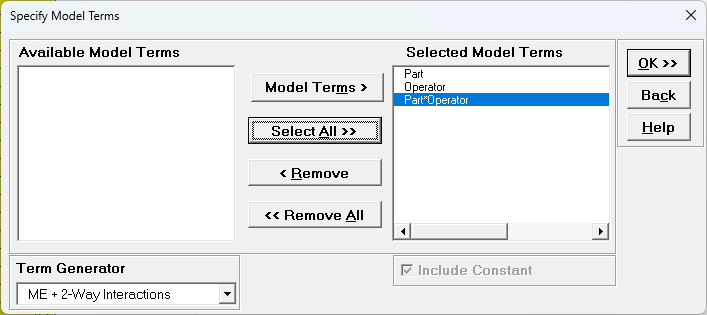5. Click OK >>. The Variance Components report is given: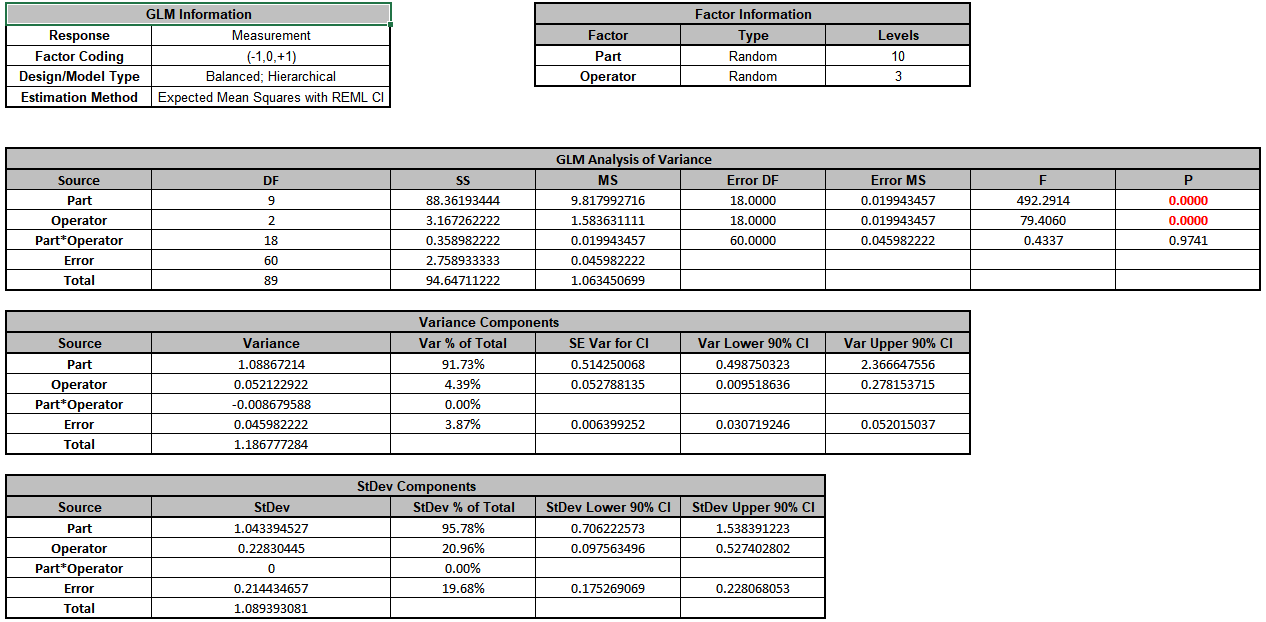6. Since the Part*Operator interaction term is not significant (alpha = 0.1), we will refit the model excluding this term. Press F3 or click Recall SigmaXL Dialog to recall last dialog.

7. Click Next >>. Select Part*Operator, click < Remove.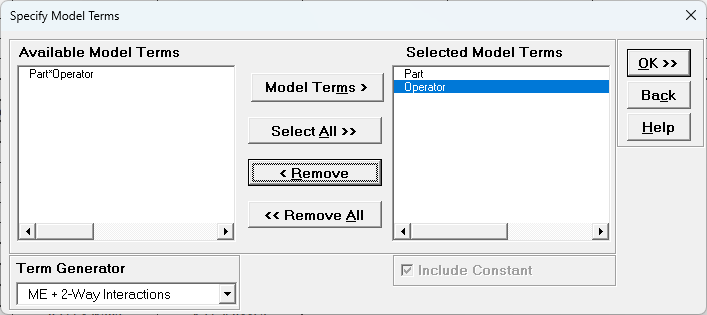8. Click OK>>. The revised Variance Components report is shown: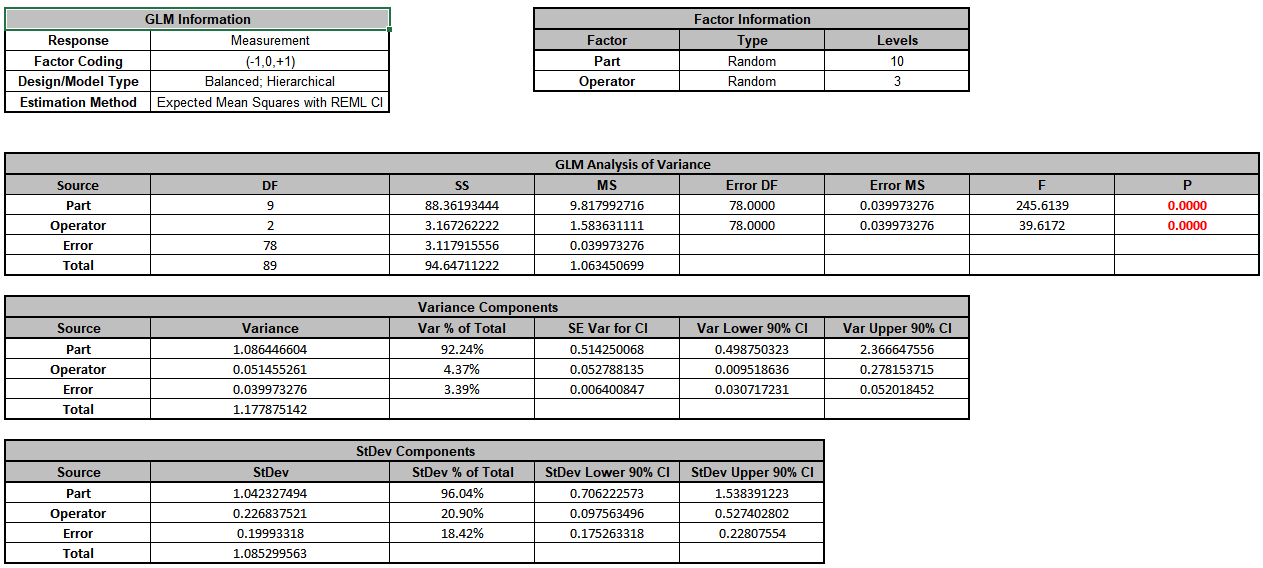9. Now we will now convert the GLM Variance Components to Gage R&R Metrics. Click on SigmaXL > Measurement Systems Analysis > Basic MSA Templates > GLM GageRR (Crossed) Metrics without Interaction to open the conversion template.

10. Copy the Variance Component values in cells C18:C20 (Sheet GLM2 – VarComp) and paste the values into the yellow highlighted cells C15:C17 of the template. For Gage Name, enter the information as shown. Enter Process Tolerance =8, use Standard Deviation Multiplier = 6.Warning: It is crucial to ensure that the GLM Variance Components Source Names match those given in the template. If the GLM model order was different than that of the template, each entry would have to be copy/pasted individually.

11. This template converts the GLM Variance Components to Gage R&R metrics. The results match those given in the original analysis, excluding the confidence intervals and ANOVA table. With %Total Variation and %Tolerance less than 30% but greater than 10%, this is a marginal measurement system.

12. Next, we will show examples where the classical Gage R&R Analysis does not work and GLM is required to do the analysis.

# Example 4: Gage R&R Study with Operator as Fixed Factor

We will reanalyze the AIAG Gage R&R data above, but now we will treat Operator as a Fixed Factor. In this case Operator could denote a test fixture and there are only three of them in the plant.

1. Open the file Gage RR - AIAG.xlsx. Preselect the worksheet data including column headings. Click SigmaXL > Statistical Tools > General Linear Model > Fit General Linear Model. Click Next>>.

2. Select Measurement, click Numeric Response (Y) >>, select Operator, click Fixed Factors - Categorical (X) >>, select Part, click Random Factors - Categorical (X) >>. Leave Nesting unchecked. We will use the default Coding for Categorical Predictors (-1, 0, +1) and default Confidence Level = 95.0%. Leave Residual Plots, Main Effects Plots, Interaction Plots and Box-Cox Transformation unchecked. Advanced Options are not available with Random Factors.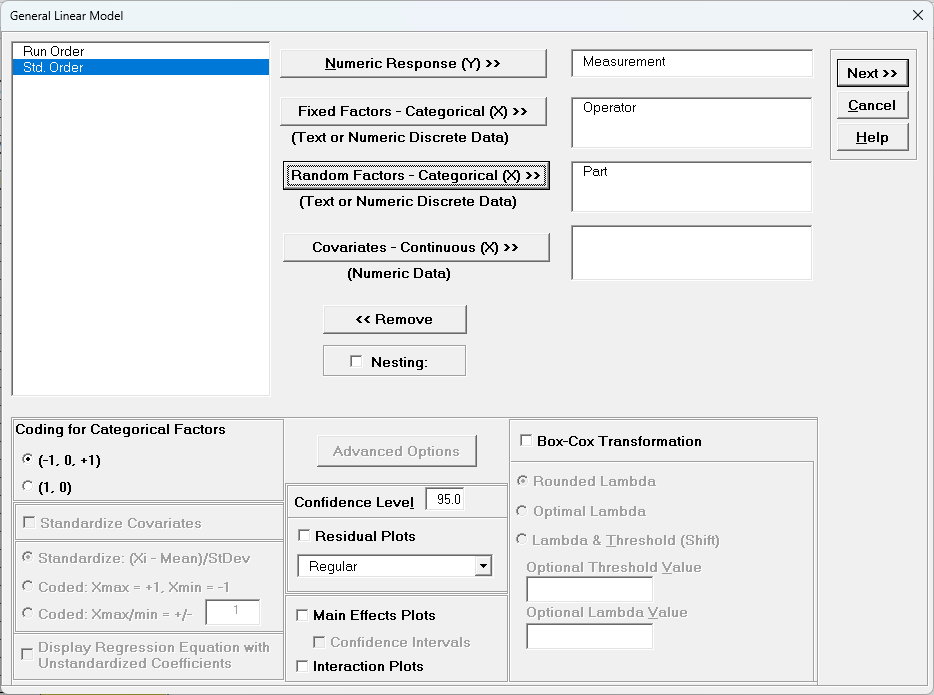3. Click Next >>.

4. Leave Term Generator as Main Effects. Click Select ALL >>.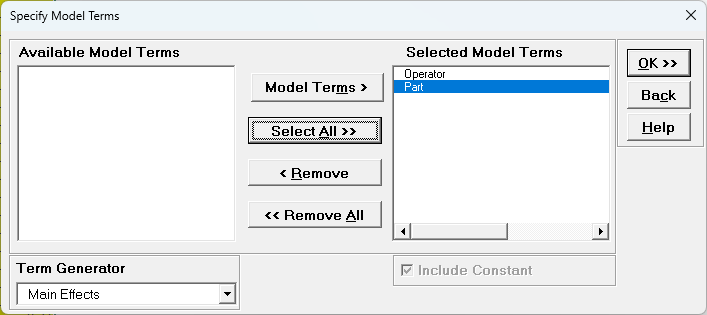5. Click OK >>. The Variance Components report is given:Note that Operator is no longer in the Variance Components table. We will need to manually calculate the VC value to be entered into the GLM GageRR template.

6. Click on Sheet GLM# Model for the current model. The Parameter Estimates are given as:7. The coded (-1, 0, 1) coefficients for Operator will be used to estimate the Fixed Factor Variance Component. It is the average of the coefficients squared, including the hidden reference value. (This is from Formula 6.2 in Burdick, R. K., Borror, C. M., and Montgomery, D. C., Design and Analysis of Gauge R&R Studies: Making Decisions with Confidence Intervals in Random and Mixed ANOVA Models, ASA-SIAM Series on Statistics and Applied Probability, 2005.) Note, this calculation cannot be done with coded (0, 1) coefficients.

Operator C coefficient (hidden reference) = -(0.18889 + 0.06689) = - 0.25578

Fixed Operator VC =((0.18889)^2 + (0.06689)^2 + (-0.25578)^2)/3 = 0.035192.

Use Excel formulas to calculate this to full precision.

8. Now we will now convert the GLM and Manual Variance Components to Gage R&R Metrics. Click on SigmaXL > Measurement Systems Analysis > Basic MSA Templates > GLM GageRR (Crossed) Metrics without Interaction to open the conversion template.

9. Enter the Variance Component values into the yellow highlighted cells C15:C17 of the template as shown. For Gage Name, enter the information as shown. Enter Process Tolerance =8, use Standard Deviation Multiplier = 6.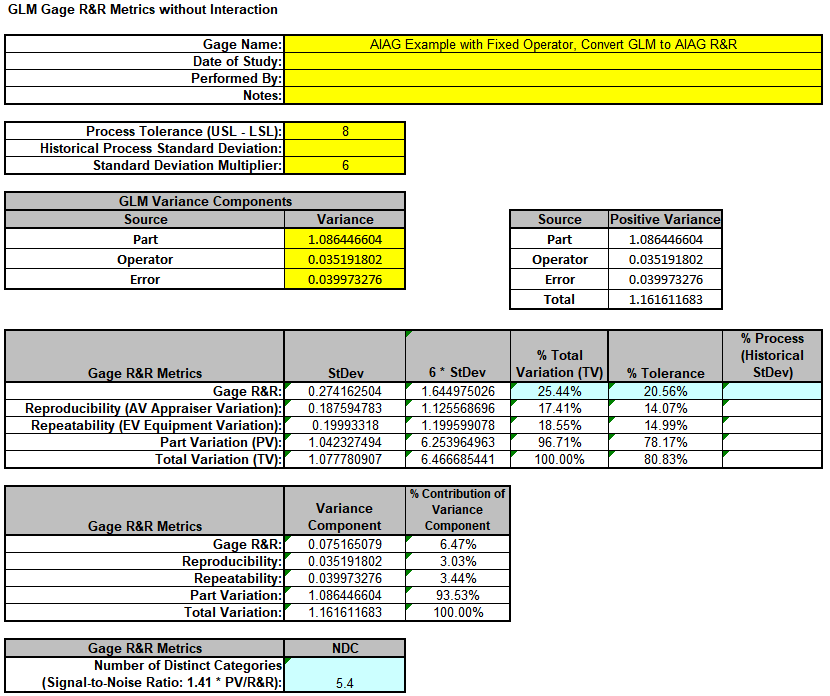Warning: It is crucial to ensure that the GLM Variance Components Source Names match those given in the template.

10. This template converts the GLM Variance Components to Gage R&R metrics. Note that the %Total Variation has been reduced from the original 27.86% to 25.44%, %Tolerance from 22.68% to 20.56% and NDC increased from 4.9 to 5.4. However, it is still considered a marginal measurement system. Note that these steps should only be done when Operator is a fixed factor.

# Example 5: Gage R&R Study with Operator and One Part

We will reanalyze the AIAG Gage R&R data, but now we will consider the case where there is only one part, i.e., like a Type 1 Gage R&R Study but with multiple operators.

1. Open the file Gage RR – AIAG.xlsx. Preselect the worksheet data including column headings. Click Excel > Data > Filter, select Part, uncheck Select All, check Part 01 as shown.

2.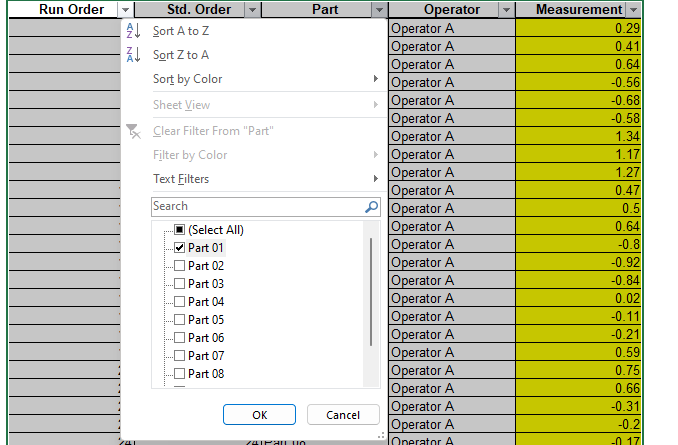3. Click OK. This hides the rows for Parts 02 to 10.

4.Note: Three measurement readings per operator for a study with one part is not recommended. We are using this example with the AIAG data for convenience.

5. Click SigmaXL > Statistical Tools > General Linear Model > Fit General Linear Model. Click Next >>.

6. Select Measurement, click Numeric Response (Y) >>, select Operator, click Random Factors - Categorical (X) >>. Leave Nesting unchecked. We will use the default Coding for Categorical Predictors (-1, 0, +1) and default Confidence Level = 95.0%. Leave Residual Plots, Main Effects Plots, Interaction Plots and Box-Cox Transformation unchecked. Advanced Options are not available with Random Factors.

7.8. Click Next >>.

9. Click Model Terms >>.

10.11. Click OK >>. The Variance Components report is given:

12.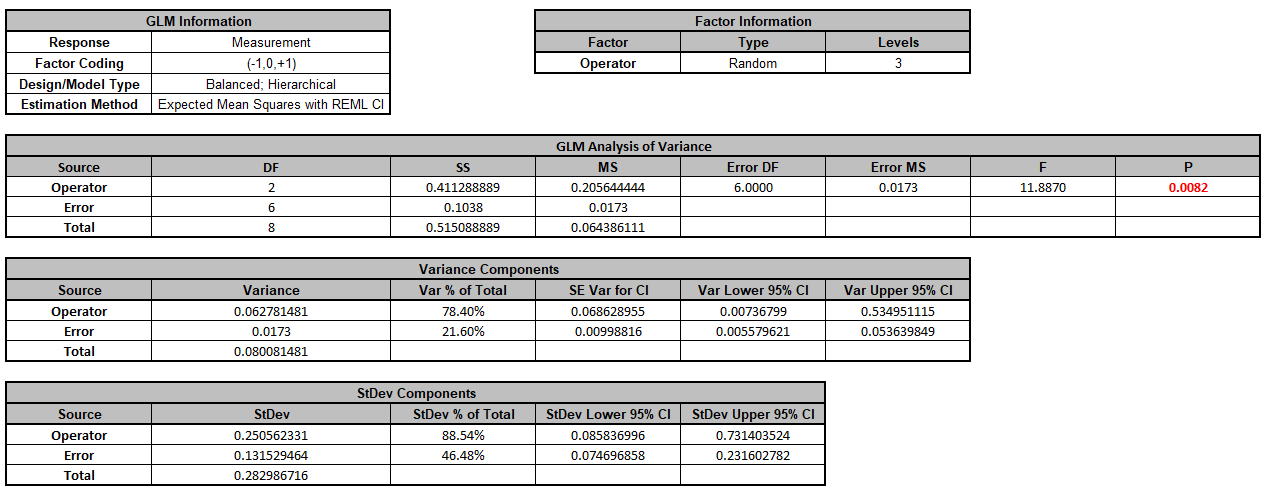13. Now we will now convert the GLM and Manual Variance Components to Gage R&R Metrics. Click SigmaXL > Measurement Systems Analysis > Basic MSA Templates > GLM GageRR (Crossed) Metrics without Interaction to open the conversion template.

14. Copy the Variance Component values in cells C16:C17 (Sheet GLM# – VarComp) and paste the values into the yellow highlighted cells C16:C17 of the template. Enter 0 for Part Variance. For Gage Name, enter the information as shown. Enter Process Tolerance =8, use Standard Deviation Multiplier = 6.

15.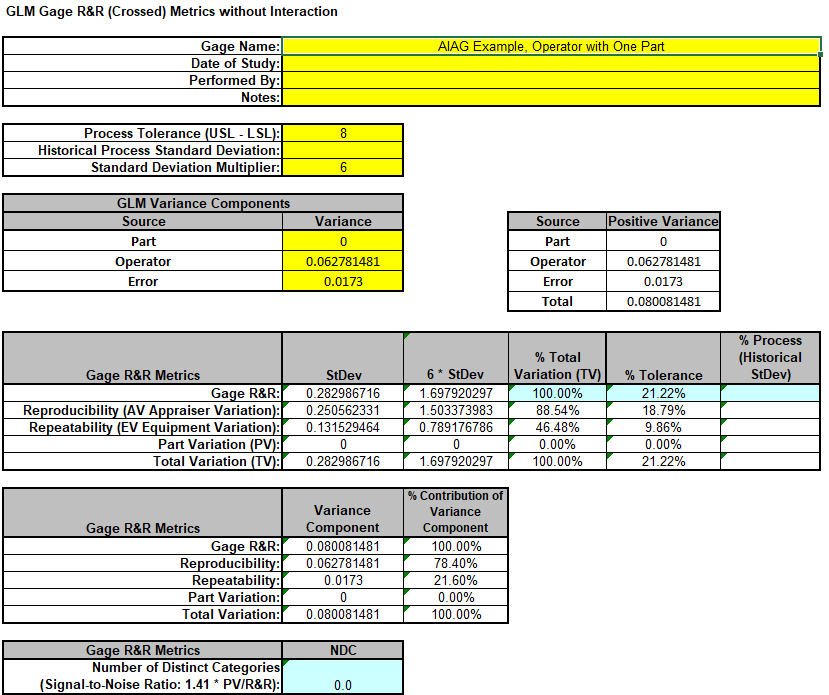Warning: It is crucial to ensure that the GLM Variance Components Source Names match those given in the template.

16. This template converts the GLM Variance Components to Gage R&R metrics. The %Total Variation is not applicable here because there is no part variation. With %Tolerance less than 30% but greater than 10%, this is a marginal measurement system.

# Example 6: Destructive (Nested) Gage R&R

We will now analyze data from a Destructive (Nested) Gage R&R found in the paper referenced below with link to the PDF. This is a shear test for a resistance spot welding process. Design of Experiments were used to produce the parts by varying preheat temperature, preheat current, welding temperature and welding current. Each run of a 4 Factor 8-Run Fractional Factorial DOE is a Part and this was replicated 6 times for a total of 48 welds. Two Shear Test machines were used so each machine tested 8 Parts with 3 Replicates. The shear test machines are the Operator and treated as a random factor in the study. Tensile Shear Strength (TSS Newtons) and Ultimate Strain (US mm) were measured, but we will only consider TSS here.

Reference: Almeida, F., Gomes, G., Sabioni, R., Gomes, J., de Paula, V., de Paiva, A., & da Costa, S. (2018)."A Gage Study Applied in Shear Test to Identify Variation Causes from a Resistance Spot Welding Measurement System", Strojni'kivestnik - Journal of Mechanical Engineering, 64(10), 621-631. https://www.sv-jme.eu/?ns_articles_pdf=/ns_articles/files/ojs/5235/public/5235-30318-1-PB.pdf&id=6160

1. Open the file Tensile Shear Strength.xlsx. Click SigmaXL > Statistical Tools > General Linear Model > Fit General Linear Model. If necessary, click Use Entire Data Table, click Next.

2. Select TSS, click Numeric Response (Y) >>, select Operator, click Random Factors - Categorical (X) >>, select Part, click Random Factors - Categorical (X) >>. Check Nesting. For the left-side drop-down Select a Factor or Covariate, select Part. For the right-side drop-down Select a Factor to Nest in:, select (Operator). We will use the default Coding for Categorical Predictors (-1, 0, +1) and default Confidence Level = 95.0%. Leave Plots, Main Effects Plots, Interaction Plots and Box-Cox Transformation unchecked. Advanced Options are not available with Random Factors.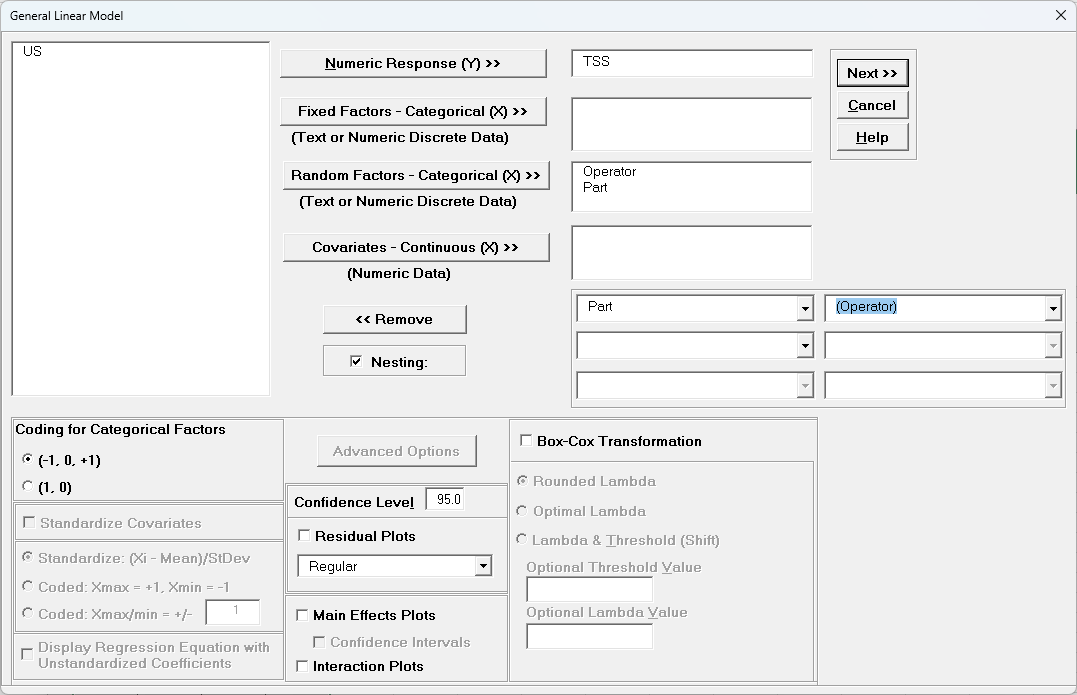3. Click Next >>.

4. Leave Term Generator as Main Effects. Click Select ALL >>.The term Part(Operator) denotes that Part is nested within Operator. Since Operator is the top level of the nesting, we place it in the model first.

5. Click OK >>. The Variance Components report is given: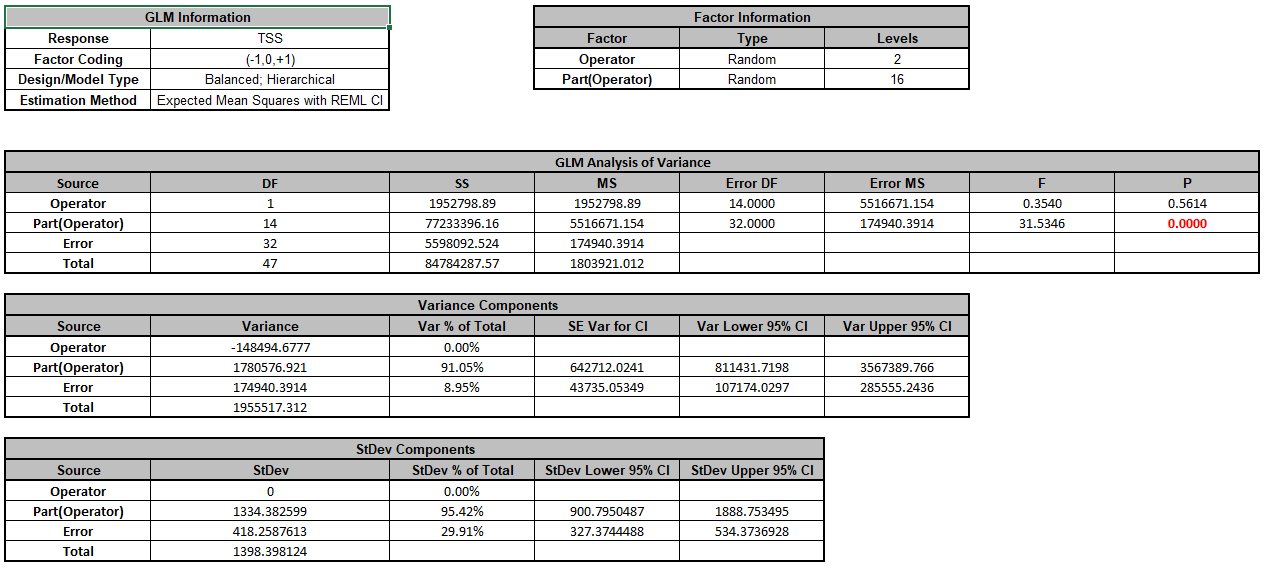Note that Operator is a negative variance component. It will be treated as zero.

6. Now we will now convert the GLM Variance Components to Gage R&R Metrics. Click on SigmaXL > Measurement Systems Analysis > Basic MSA Templates > GLM GageRR (Nested) Metrics to open the conversion template.

7. Copy the Variance Component values in cells C18:C20 (Sheet GLM1 - VarComp) and paste the values into the yellow highlighted cells C15:C17 of the template. For Gage Name, enter the information as shown. Use Standard Deviation Multiplier = 6.Warning: It is crucial to ensure that the GLM Variance Components Source Names match those given in the template. If the GLM model order was different than that of the template, each entry would have to be copy/pasted individually.

8. This template converts the GLM Variance Components to Gage R&R metrics. With %Total Variation less than 30% but greater than 10%, this is a marginal measurement system. The results match those given in the paper.

# Example 7: Expanded Gage R&R

We will now analyze data from an Expanded Gage R&R found in the paper referenced below with link to the PDF. This is a wafer thickness measurement (Angstroms) for a semiconductor process. The design includes random factors: Batch, Wafer, Location and Operator. Wafer is nested within Batch and Location is nested within Wafer. There are 3 Batches, 3 Wafers, 4 Locations, 3 Operators and 2 Replicates for a total of 216 thickness measurements. The variance components for Part/Process include Batch, Wafer(Batch) and Location(Wafer(Batch)). The variance components for Reproducibility include Operator, Batch*Operator, Wafer(Batch)*Operator and Location(Wafer(Batch))*Operator. The variance component for Repeatability is the Error term from Replicates.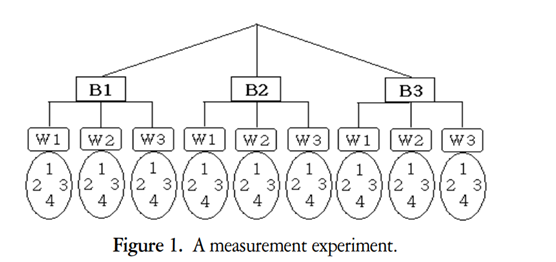Reference: Lee, S.H., Lee, C.W. (2005), A Study of Gage R&R Analysis Considering the Variations of Between-Within Group and Within Part IE Interfaces 18(4), 444-453. https://koreascience.kr/article/JAKO200529256810322.pdf. Written in Korean but abstract, formulas, figures and tables are in English.

1. Open the file Wafer Thickness.xlsx. Click SigmaXL > Statistical Tools > General Linear Model > Fit General Linear Model. If necessary, click Use Entire Data Table, click Next.

2. Select Thickness, click Numeric Response (Y) >>, select Batch to Operator, click Random Factors - Categorical (X) >>. Check Nesting. For the left-side drop-down Select a Factor or Covariate, select Wafer. For the right-side drop-down Select a Factor to Nest in:, select (Batch). For the second left-side drop-down Select a Factor or Covariate, select Location. For the right-side drop-down Select a Factor to Nest in:, select (Wafer). We will use the default Coding for Categorical Predictors (-1, 0, +1) and default Confidence Level = 95.0%. Leave Residual Plots, Main Effects Plots, Interaction Plots and Box-Cox Transformation unchecked. Advanced Options are not available with Random Factors.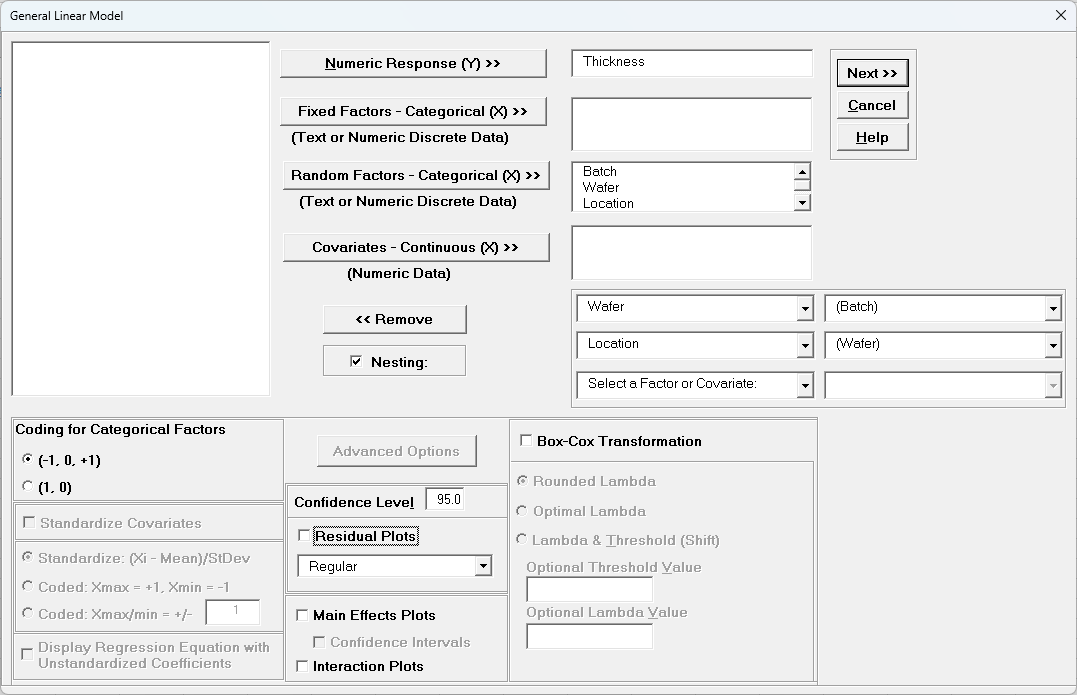3. Click Next >>.

4. Using Term Generator, select ME + 2-Way Interactions. Click Select ALL >>.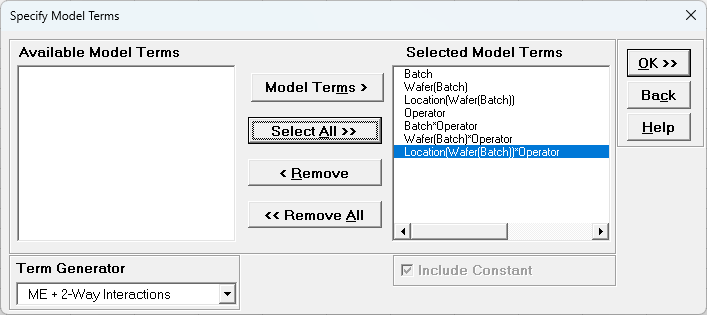The term Wafer(Batch) denotes that Wafer is nested within Batch. The term Location(Wafer(Batch)) denotes that Location is nested within Wafer which is nested within Batch. Only legal 2-Way Interactions are available, so Wafer*Batch, Location*Wafer or Location*Batch are not available.

5. Click OK >>. The Variance Components report is given: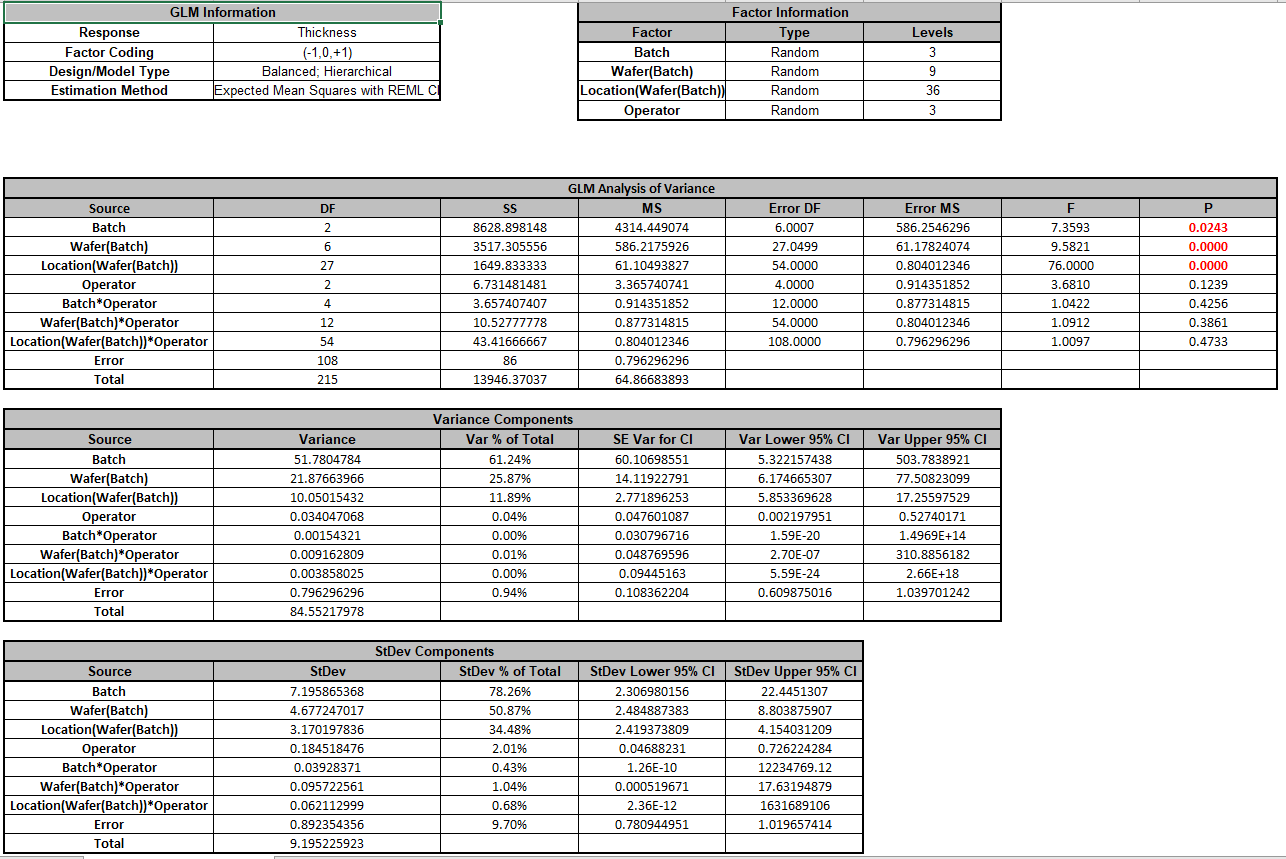Note that the variance component confidence intervals for the interaction terms with Operator are very wide and the P-Values are insignificant (alpha = 0.05), so the model could be refit excluding these terms but we will not do so here.

6. Now we will now convert the GLM Variance Components to Gage R&R Metrics. Click on SigmaXL > Measurement Systems Analysis > Basic MSA Templates > GLM GageRR (Expanded) Metrics to open the conversion template.

7. Copy the Variance Component values in Sheet GLM1 - VarComp and paste the values into the yellow highlighted cells of the template as shown. For Gage Name, enter the information as shown. Use Standard Deviation Multiplier = 6.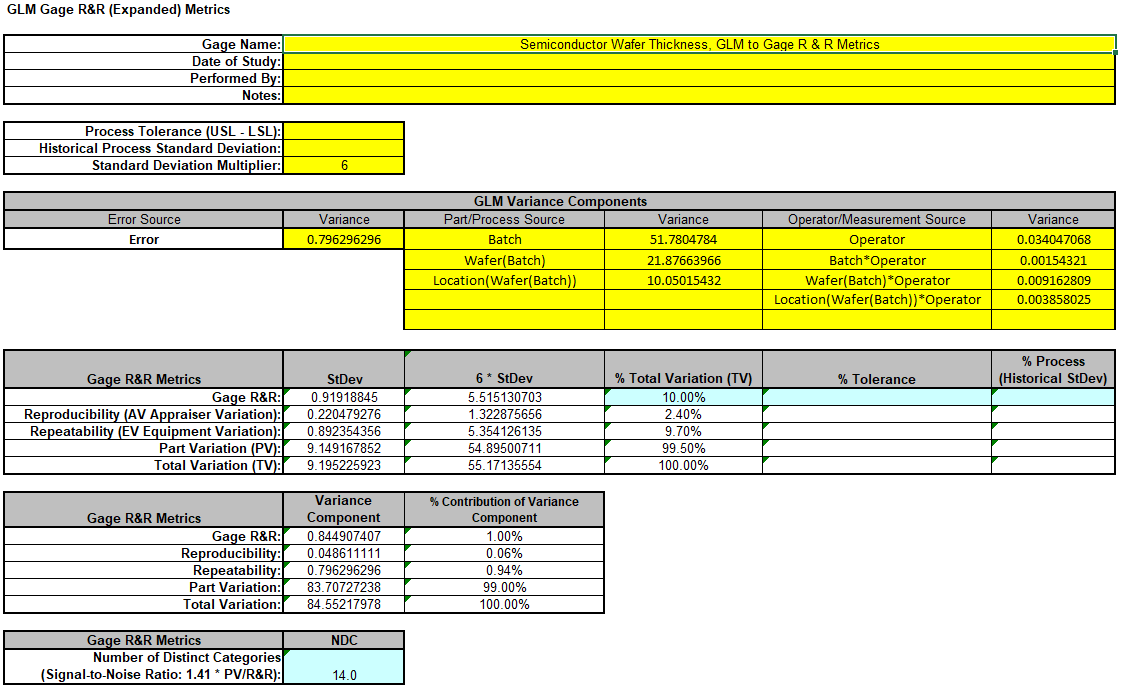8. This template converts the GLM Variance Components to Gage R&R metrics. With %Total Variation = 10%, this is an acceptable measurement system. The results match those given in the paper.

# Example 8: Unbalanced Nested Factorial Experiment with Fixed and Random Factors

We will now analyze a nested factorial experiment adapted from Example 14.2 in Montgomery's Design and Analysis of Experiments book. The process is the hand insertion of electronic components on printed circuit boards and the goal of the study is to improve the speed of the assembly operation. The design includes three assembly fixtures and two workplace layouts. Operators are required to perform the assembly.

In the book, four operators are randomly selected for each fixture-layout combination, but here we will use 4 operators for layout 1, but three for layout 2 making it an unbalanced design. The operators chosen for layout 1 are different individuals from those chosen for layout 2, so operators are nested within layout. Because there are only three fixtures and two layouts, these are fixed factors but the operators are random factors, so this is a mixed model. The treatment combinations in this design are run in random order, but the worksheet is shown in standard order. Two replicates are obtained. The assembly times are measured in seconds.

Since the design is unbalanced, SigmaXL will automatically use Restricted Maximum Likelihood (REML) to calculate variance components rather than the traditional ANOVA Expected Means Squares (EMS) method

Reference: Montgomery, D.C. (2020). Design and Analysis of Experiments, 10th Edition, John Wiley & Sons.

1. Open the file Assembly Time Nested Factorial.xlsx. Click SigmaXL > Statistical Tools > General Linear Model > Fit General Linear Model. If necessary, click Use Entire Data Table, click Next.

2. Select Time, click Numeric Response (Y) >>, select Fixture and Layout, click Fixed Factors - Categorical (X) >>, select Operator, click Random Factors - Categorical (X) >>. Check Nesting. For the left-side drop-down Select a Factor or Covariate, select Operator. For the right-side drop-down Select a Factor to Nest in:, select (Layout). We will use the default Coding for Categorical Predictors (-1, 0, +1) and default Confidence Level = 95.0%. Check Main Effects Plots and Interaction Plots. Leave Residual Plots, and Box-Cox Transformation unchecked. Advanced Options are not available with Random Factors.3. Click Next >>.

4. Using Term Generator, select ME + 2-Way Interactions. Click Select ALL >>.The term Operator(Layout) denotes that Operator is nested within Layout. Only legal 2-Way Interactions are available, so Operator*Layout is not available.

5. Click OK >>. The Variance Components report is given: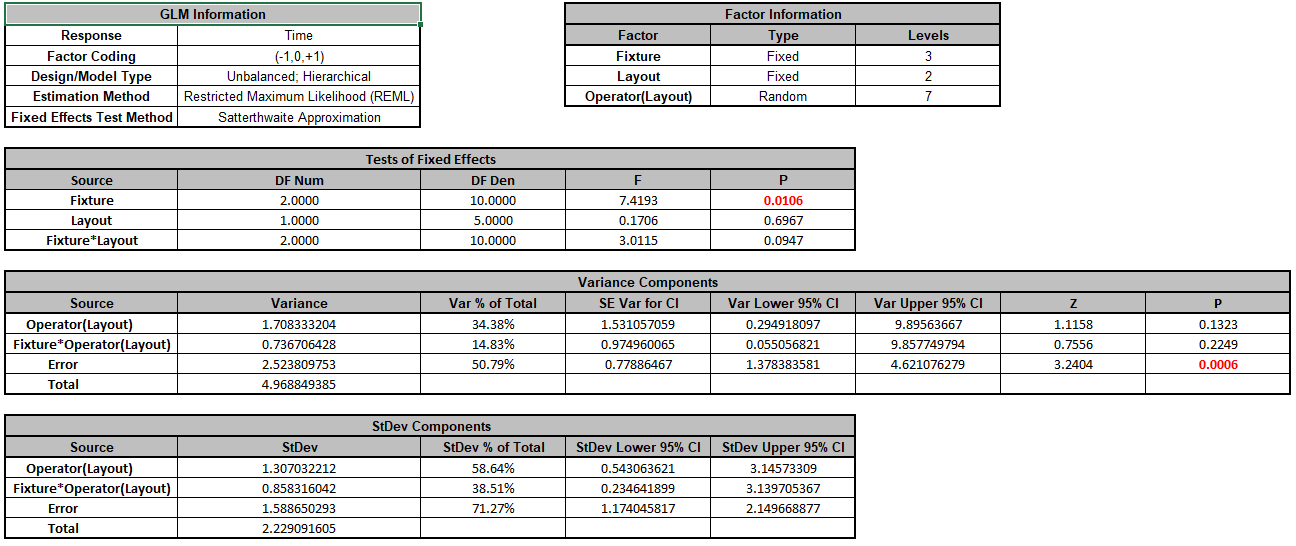Since this is an unbalanced design, Restricted Maximum Likelihood (REML) is used to estimate the variance components. In Tests of Fixed Effects, we see that Fixture is significant but Layout and Fixture*Layout are not significant. In Variance Components, Operator(Layout) contributes to 34.4% of the total variance and the interaction Fixture*Operator(Layout) contributes to 14.8% of the total variance. The P-Values for these variance components are not significant but this test is known to be underpowered when there are only a few levels (see Appendix Random Factors).

6. Click Sheet GLM1 - Plots to view the Main Effects and Interaction Plots.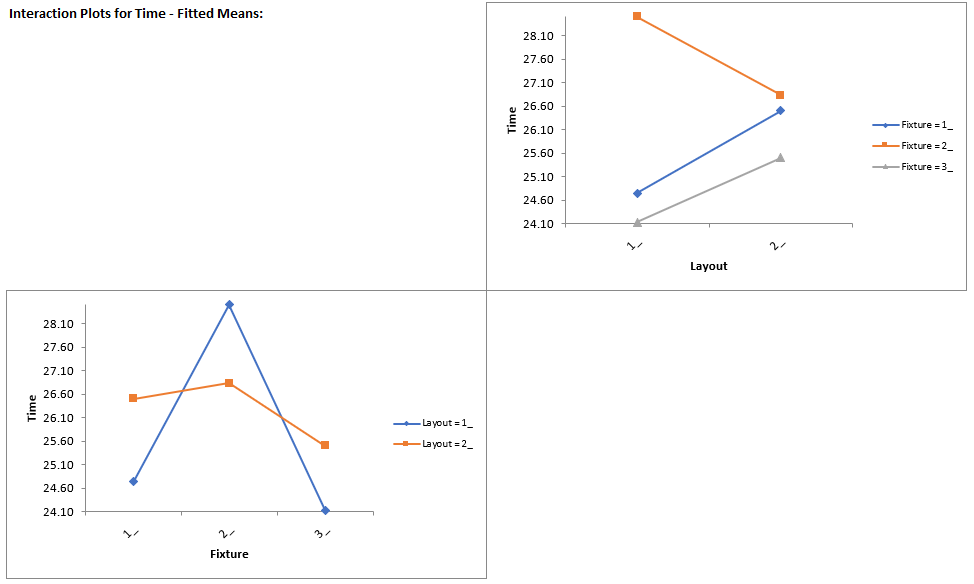Clearly Fixture 2 results in an increase in assembly time. This may be an operator training issue but possibly the fixture can be modified to help the operators perform the assembly task more quickly.

Note: The Main Effects and Interaction Plots are computed from the predicted values of the regression model which assumes that all factors are fixed. Confidence Intervals for the Main Effects plots are not available when there is a Random Factor.

# Web Demos

Our CTO and Co-Founder, John Noguera, regularly hosts free Web Demos featuring SigmaXL and DiscoverSim